FreeFEM Documentation on GitHub

stars - forks

# Finite element

As stated in tutorials, FEM approximates all functions $$w$$ as:

$w(x,y)\simeq w_0\phi_0(x,y)+w_1\phi_1(x,y)+\cdots+w_{M-1}\phi_{M-1}(x,y)$

with finite element basis functions $$\phi_k(x,y)$$ and numbers $$w_k$$ ($$k=0,\cdots,M-1$$). The functions $$\phi_k(x,y)$$ are constructed from the triangle $$T_{i_k}$$, and called shape functions.

In FreeFEM, the finite element space:

$V_h=\left\{w\left|\; w_0\phi_0+w_1\phi_1+\cdots+w_{M-1}\phi_{M-1},\, w_i\in \R\right.\right\}$

is easily created by

• in 2d
 1 fespace IDspace(IDmesh,); 

or with $$\ell$$ pairs of periodic boundary conditions in 2D:

 1 2 3 4 fespace IDspace(IDmesh,, periodic=[[la1, sa1], [lb1, sb1], ... [lak, sak], [lbk, sbl]]); 
• in 3D:
 1 2 3 4 fespace IDspace(IDmesh3,, periodic=[[la1, sa1, ta1], [lb1, sb1, tb1], ... [lak, sak, tak], [lbk, sbl, tbl]]); 
• in surface 3D:
 1 2 3 4 fespace IDspace(IDmeshS,, periodic=[[la1, sa1, ta1], [lb1, sb1, tb1], ... [lak, sak, tak], [lbk, sbl, tbl]]); 

where IDspace is the name of the space (e.g. Vh), IDmesh IDmesh3 IDmeshS is respectly the name of the associated :freefem:mesh, mesh3, meshS and <IDFE> is an identifier of finite element type.

In 2D we have a pair of periodic boundary conditions, if $$[la_i, sa_i]$$, $$[lb_i, sb_i]$$ is a pair of int, and the 2 labels $$la_i$$ and $$lb_i$$ refer to 2 pieces of boundary to be in equivalence.

If $$[la_i, sa_i]$$, $$[lb_i, sb_i]$$ is a pair of real, then $$sa_i$$ and $$sb_i$$ give two common abscissa on the two boundary curves, and two points are identified as one if the two abscissa are equal.

In 2D, we have a pair of periodic boundary conditions, if $$[la_i, sa_i, ta_i]$$, $$[lb_i, sb_i, tb_i]$$ is a pair of int, the 2 labels $$la_i$$ and $$lb_i$$ define the 2 pieces of boundary to be in equivalence.

If $$[la_i, sa_i, ta_i]$$, $$[lb_i, sb_i, tb_i]$$ is a pair of real, then $$sa_i$$, $$ta_i$$ and $$sb_i$$, $$tb_i$$ give two common parameters on the two boundary surfaces, and two points are identified as one if the two parameters are equal.

Note

The 2D mesh of the two identified borders must be the same, so to be sure, use the parameter fixedborder=true in buildmesh command (see fixedborder).

## List of the types of finite elements

As of today, the known types of finite elements are:

• [P0] piecewise constant discontinuous finite element (2d, 3d, surface 3d), the degrees of freedom are the barycenter element value.

(9) $\P^0_{h} = \left\{ v \in L^2(\Omega) \left|\; \textrm{for all }K \in \mathcal{T}_{h}\;\;\textrm{there is }\alpha_{K}\in \R : \;\; v_{|K} = \alpha_{K } \right.\right\}$
• [P1] piecewise linear continuous finite element (2d, 3d, surface 3d), the degrees of freedom are the vertices values.

(10) $\P^1_{h} = \left\{ v \in H^{1}(\Omega) \left|\; \forall K \in \mathcal{T}_{h},\ v_{|K} \in P_{1} \right.\right\}$
• [P1dc] piecewise linear discontinuous finite element (2d, 3d with load”Element_P1dc1”)

(11) $\P^1_{dc|h} = \left\{ v \in L^{2}(\Omega) \left|\; \forall K \in \mathcal{T}_{h}, \ v_{|K} \in P_{1} \right.\right\}$

Warning

Due to an interpolation problem, the degree of freedom is not the vertices but three vertices which move inside $$T(X)= G + .99 (X-G)$$ where $$G$$ is the barycenter.

• [P1b] piecewise linear continuous finite element plus bubble (2d, 3d)

The 2D Case:

(12) $\P^1_{b|h} = \left\{ v \in H^{1}(\Omega) \left|\; \forall K \in \mathcal{T}_{h}, \ v_{|K} \in P_{1} \oplus \mathrm{Span}\{ \lambda^{K}_{0} \lambda^{K}_{1} \lambda^{K}_{2} \} \right.\right\}$

The 3D Case:

(13) $\P^1_{b|h} = \left\{ v \in H^{1}(\Omega) \left|\; \forall K \in \mathcal{T}_{h}, \ v_{|K} \in P_{1} \oplus \mathrm{Span}\{ \lambda^{K}_{0} \lambda^{K}_{1} \lambda^{K}_{2} \lambda^{K}_{3} \} \right.\right\}$

where $$\lambda^{K}_{i}, i=0,..,d$$ are the $$d+1$$ barycentric coordinate functions of the element $$K$$ (triangle or tetrahedron).

• P1bl,P1bl3d piecewise linear continuous finite element plus linear bubble (with load”Element_P1bl” 2d, 3d).

The bubble is built by splitting the $$K$$, a barycenter in $$d+1$$ sub element. (need load "Element_P1bl")

• [P2, P2] piecewise $$P_{2}$$ continuous finite element (2d, 3d, surface 3d)

$\P^2_{h} = \left\{ v \in H^{1}(\Omega) \left|\; \forall K \in \mathcal{T}_{h}, \ v_{|K} \in P_{2} \right.\right\}$

where $$P_{2}$$ is the set of polynomials of $$\R^{2}$$ of degrees $$\le 2$$.

• [P2b, P2b3d] piecewise $$P_{2}$$ continuous finite element plus bubble (2d, 3d with load”Element_P2bulle3”)

The 2D Case:

$\P^2_{h} = \left\{ v \in H^{1}(\Omega) \left|\; \forall K \in \mathcal{T}_{h}, \ v_{|K} \in P_{2} \oplus \mathrm{Span}\{ \lambda^{K}_{0} \lambda^{K}_{1} \lambda^{K}_{2} \} \right.\right\}$

The 3D Case:

$\P^2_{h} = \left\{ v \in H^{1}(\Omega) \left|\; \forall K \in \mathcal{T}_{h}, \ v_{|K} \in P_{2} \oplus \mathrm{Span}\{ \lambda^{K}_{0} \lambda^{K}_{1} \lambda^{K}_{2} \lambda^{K}_{3} \} \right.\right\}$
• [P2dc] piecewise $$P_{2}$$ discontinuous finite element (2d)

$\P^2_{dc|h} = \left\{ v \in L^{2}(\Omega) \left|\; \forall K \in \mathcal{T}_{h}, \ v_{|K} \in P_{2} \right.\right\}$

Warning

Due to an interpolation problem, the degree of freedom is not the six P2 nodes but six nodes which move inside $$T(X)= G + .99 (X-G)$$ where $$G$$ is the barycenter.

• [P2h] quadratic homogeneous continuous (without P1).

• [P3] piecewise $$P_{3}$$ continuous finite element (2d) (needs load "Element_P3")

$\P^3_{h} = \left\{ v \in H^{1}(\Omega) \left|\; \forall K \in \mathcal{T}_{h}, \ v_{|K} \in P_{3} \right.\right\}$

where $$P_{3}$$ is the set of polynomials of $$\R^{2}$$ of degrees $$\le 3$$.

• [P3dc] piecewise $$P_{3}$$ discontinuous finite element (2d) (needs load "Element_P3dc")

$\P^3_{dc|h} = \left\{ v \in L^2(\Omega) \left|\; \forall K \in \mathcal{T}_{h}, \ v_{|K} \in P_{3} \right.\right\}$

where $$P_{3}$$ is the set of polynomials of $$\R^{2}$$ of degrees $$\le 3$$.

• [P4] piecewise $$P_{4}$$ continuous finite element (2d) (needs load "Element_P4")

$\P^4_{h} = \left\{ v \in H^{1}(\Omega) \left|\; \forall K \in \mathcal{T}_{h},\ v_{|K} \in P_{4} \right.\right\}$

where $$P_{4}$$ is the set of polynomials of $$\R^{2}$$ of degrees $$\le 4$$.

• [P4dc] piecewise $$P_{4}$$ discontinuous finite element (2d) (needs load "Element_P4dc")

$\P^4_{dc|h} = \left\{ v \in L^2(\Omega) \left|\; \forall K \in \mathcal{T}_{h}, \ v_{|K} \in P_{3} \right.\right\}$

where $$P_{4}$$ is the set of polynomials of $$\R^{2}$$ of degrees $$\le 3$$.

• [P0Edge] piecewise $$P_{0}$$ discontinuous finite element (2d) contained on each edge of the mesh.

• [P1Edge] piecewise $$P_{1}$$ discontinuous finite element (2d) (needs load "Element_PkEdge") $$P_1$$ on each edge of the mesh.

• [P2Edge] piecewise $$P_{2}$$ discontinuous finite element (2d) (needs load "Element_PkEdge") $$P_2$$ on each edge of the mesh.

• [P3Edge] piecewise $$P_{3}$$ discontinuous finite element (2d) (needs load "Element_PkEdge") $$P_3$$ on each edge of the mesh.

• [P4Edge] piecewise $$P_{4}$$ discontinuous finite element (2d) (needs load "Element_PkEdge") $$P_4$$ on each edge of the mesh.

• [P5Edge] piecewise $$P_{5}$$ discontinuous finite element (2d) (needs load "Element_PkEdge") $$P_5$$ on each edge of the mesh.

• [P2Morley] piecewise $$P_{2}$$ non conform finite element (2d) (needs load "Morley")

$\begin{split}\P^2_{h} = \left\{ v \in L^2(\Omega) \left|\; \forall K \in \mathcal{T}_{h}, \ v_{|K} \in P_{3}, \left\{\begin{array}{c} v \mbox{ continuous at vertices,}\\ \p_n{v} \mbox{ continuous at middle of edge,} \end{array}\right. \right.\right\}\end{split}$

where $$P_{2}$$ is the set of polynomials of $$\R^{2}$$ of degrees $$\le 2$$.

Warning

To build the interplant of a function $$u$$ (scalar) for this finite element, we need the function and 2 partial derivatives $$(u,u_x, u_y)$$, creating this vectorial finite element with 3 components $$(u,u_x,u_y)$$.

See our example for solving the BiLaplacien problem:

  1 2 3 4 5 6 7 8 9 10 11 12 13 14 15 16 17 18 19 20 21 22 23 24 25 26 27 28 29 30 31 32 load "Morley" // Parameters int nn = 10; real h = 0.01; real f = 1; // Mesh mesh Th = square(nn, nn); Th = adaptmesh(Th, h, IsMetric=1); // Fespace fespace Vh(Th, P2Morley); //The Morley finite element space Vh [u, ux, uy], [v, vx, vy]; // Macro macro bilaplacien(u, v) (dxx(u)*dxx(v) + dyy(u)*dyy(v) + 2.*dxy(u)*dxy(v)) // // Problem solve bilap ([u, ux, uy], [v, vx, vy]) = int2d(Th)( bilaplacien(u, v) ) - int2d(Th)( f*v ) + on(1, 2, 3, 4, u=0, ux=0, uy=0) ; // Plot plot(u, cmm="u"); 
• [HCT] $$P_3$$ $$C^1$$ conforms finite element (2d) (needs load "Element_HCT") one 3 sub triangles.

Lets call $$\mathcal{T}^\triangle_{h}$$ the sub mesh of $$\mathcal{T}_{h}$$ where all triangles are split in 3 at the barycenter.

$\P^{HCT}_{h} = \left\{ v \in C^1(\Omega) \left|\; \forall K \in \mathcal{T}^\triangle_{h}, \ v_{|K} \in P_{3} \right.\right\}$

where $$P_{3}$$ is the set of polynomials of $$\R^{2}$$ of degrees $$\le 3$$.

The degrees of freedom are the values of the normal derivative at the mid-point of each edge [BERNADOU1980].

Warning

To build the interplant of a function $$u$$ (scalar) for this finite element, we need the function and 2 partial derivatives $$(u,u_x, u_y)$$, creating this vectorial finite element with 3 components $$(u,u_x,u_y)$$ like in previous finite element.

• [P2BR] (needs load "BernadiRaugel") the Bernadi Raugel Finite Element is a Vectorial element (2d) with 2 components, see [BERNARDI1985].

It is a 2D coupled Finite Element, where the Polynomial space is $$P_1^2$$ with 3 normal bubble edge functions $$(P_2)$$. There are 9 degrees of freedom:

• 2 components at each of the 3 vertices and
• the 3 flux on the 3 edges.
• [RT0, RT03d] Raviart-Thomas finite element of degree $$0$$.

The 2D Case:

(14) $RT0_{h} = \left\{ \mathbf{v} \in H(\textrm{div}) \left|\; \forall K \in \mathcal{T}_{h} ,\ \mathbf{v}_{|K}(x,y) = \vecttwo{\alpha^1_{K}}{\alpha^2_{K}} + \beta_{K}\vecttwo{x}{y} \right.\right\}$

The 3D Case:

(15) $RT0_{h} = \left\{ \mathbf{v} \in H(\textrm{div}) \left|\; \forall K \in \mathcal{T}_{h},\ \mathbf{v}_{|K}(x,y,z) = \vectthree{\alpha^1_{K}}{\alpha^2_{K}}{\alpha^3_{K}} + \beta_{K}\vectthree{x}{y}{z} \right.\right\}$

where by writing $$\textrm{div }\mathbf{w}=\sum_{i=1}^d\p w_i/\p x_i$$ with $$\mathbf{w}=(w_i)_{i=1}^d$$:

$H(\textrm{div})=\left\{\mathbf{w}\in L^{2}(\Omega)^d\left|\textrm{div } \mathbf{w}\in L^{2}(\Omega)\right.\right\}$

and where $$\alpha^1_{K}$$, $$\alpha^2_{K}$$, $$\alpha^3_{K}$$, $$\beta_{K}$$ are real numbers.

• [RT0Ortho] Raviart-Thomas Orthogonal, or Nedelec finite element type I of degree $$0$$ in dimension 2

(16) $RT0Ortho{h} = \left\{ \mathbf{v} \in H(\textrm{curl}) \left|\; \forall K \in \mathcal{T}_{h},\ \mathbf{v}_{|K}(x,y) = \vecttwo{\alpha^1_{K}}{\alpha^2_{K}} + \beta_{K}\vecttwo{-y}{x} \right.\right\}$
• [Edge03d] 3d Nedelec finite element or Edge Element of degree $$0$$.

$Edge0_{h} = \left\{ \mathbf{v} \in H(\textrm{Curl}) \left|\; \forall K \in\mathcal{T}_{h}, \ \mathbf{v}_{|K}(x,y,z) = \vectthree{\alpha^1_{K}}{\alpha^2_{K}}{\alpha^3_{K}} + \vectthree{\beta^1_{K}}{\beta^2_{K}}{\beta^3_{K}}\times\vectthree{x}{y}{z} \right.\right\} :label:eq:Edge03d$

where by writing $$\textrm{curl}\mathbf{w}=\vectthree{\p w_2/\p x_3-\p w_3/\p x_2}{\p w_3/\p x_1-\p w_1/\p x_3}{\p w_1/\p x_2-\p w_2/\p x_1}$$ with $$\mathbf{w}=(w_i)_{i=1}^d$$:

$H(\textrm{curl})=\left\{\mathbf{w}\in L^{2}(\Omega)^d\left|\textrm{curl } \mathbf{w}\in L^{2}(\Omega)^d\right.\right\}$

and $$\alpha^1_{K},\alpha^2_{K},\alpha^3_{K},\beta^1_{K},\beta^2_{K},\beta^3_{K}$$ are real numbers.

• [Edge13d] (needs load "Element_Mixte3d") 3d Nedelec finite element or Edge Element of degree $$1$$.

• [Edge23d] (needs load "Element_Mixte3d") 3d Nedelec finite element or Edge Element of degree $$2$$.

• [P1nc] piecewise linear element continuous at the mid-point of the edge only in 2D (Crouzeix-Raviart Finite Element 2D).

• [P2pnc] piecewise quadratic plus a P3 bubble element with the continuity of the 2 moments on each edge (needs load "Element_P2pnc")

• [RT1] (needs load "Element_Mixte")

(17) $RT1_{h} = \left\{ \mathbf{v} \in H(\textrm{div}) \left|\; \forall K \in\mathcal{T}_{h}, \ \alpha^1_{K}, \alpha^2_{K}, \beta_{K} \in P_1^2,P_0, \mathbf{v}_{|K}(x,y) = \vecttwo{\alpha^1_{K}}{\alpha^2_{K}} + \beta_{K}\vecttwo{x}{y} \right.\right\}$
• [RT1Ortho] (needs load "Element_Mixte")

(18) $RT1_{h} = \left\{ \mathbf{v} \in H(\textrm{curl}) \left|\; \forall K \in\mathcal{T}_{h},\ \alpha^1_{K}, \alpha^2_{K}, \beta_{K} \in P_1^2,P_0, \mathbf{v}_{|K}(x,y) = \vecttwo{\alpha^1_{K}}{\alpha^2_{K}} + \beta_{K}\vecttwo{-y}{x} \right.\right\}$
• [RT2] (needs load "Element_Mixte")

(19) $RT2_{h} = \left\{ \mathbf{v} \in H(\textrm{div}) \left|\; \forall K \in\mathcal{T}_{h},\ \alpha^1_{K}, \alpha^2_{K}, \beta_{K} \in P_2^2, P_1, \mathbf{v}_{|K}(x,y) = \vecttwo{\alpha^1_{K}}{\alpha^2_{K}} + \beta_{K}\vecttwo{x}{y} \right.\right\}$
• [RT2Ortho] (needs load "Element_Mixte")

(20) $RT2_{h} = \left\{ \mathbf{v} \in H(\textrm{curl}) \left|\; \forall K \in\mathcal{T}_{h} ,\ \alpha^1_{K}, \alpha^2_{K}, \beta_{K} \in P_2^2, P_1,\ \mathbf{v}_{|K}(x,y) = \vecttwo{\alpha^1_{K}}{\alpha^2_{K}} + \beta_{K}\vecttwo{-y}{x} \right.\right\}$
• [BDM1] (needs load "Element_Mixte") the Brezzi-Douglas-Marini finite element:

(21) $BDM1_{h} = \left\{ \mathbf{v} \in H(\textrm{div}) \left|\; \forall K \in\mathcal{T}_{h},\ \mathbf{v}_{|K} \in P_1^2\right.\right\}$
• [BDM1Ortho] (needs load "Element_Mixte") the Brezzi-Douglas-Marini Orthogonal also call Nedelec of type II , finite element

(22) $BDM1Ortho_{h} = \left\{ \mathbf{v} \in H(\textrm{curl}) \left|\; \forall K \in\mathcal{T}_{h},\ \mathbf{v}_{|K} \in P_1^2\right.\right\}$
• [FEQF] (needs load "Element_QF") the finite element to store functions at default quadrature points (so the quadrature is qf5pT in 2D and is qfV5 in 3d).

For over quadrature you have the following corresponding finite element’s quadrature formula.

• FEQF1 $$\mapsto$$ qf1pT,
• FEQF2 $$\mapsto$$ qf2pT,
• FEQF5 $$\mapsto$$ qf5pT,
• FEQF7 $$\mapsto$$ qf7pT,
• FEQF9 $$\mapsto$$ qf9pT,
• FEQF13d $$\mapsto$$ qfV1,
• FEQF23d $$\mapsto$$ qfV2,
• FEQF53d $$\mapsto$$ qfV5

You can use this element to optimize the storage and reuse of functions with a long formula inside an integral for non linear processes.

## Use of fespace in 2D

With the 2D finite element spaces

$X_{h} = \left\{ v \in H^{1}(]0,1[^2) |\; \forall K \in \mathcal{T}_{h}\quad v_{|K} \in P_{1} \right\}$
$X_{ph} = \left\{ v \in X_{h} |\; v\left(\vecttwo{0}{.}\right) = v\left(\vecttwo{1}{.}\right) , v\left(\vecttwo{.}{0}\right) = v\left(\vecttwo{.}{1}\right) \right\}$
$M_{h} = \left\{ v \in H^{1}(]0,1[^2) |\; \forall K \in \mathcal{T}_{h}\quad v_{|K} \in P_{2} \right\}$
$R_{h} = \left\{ \mathbf{v} \in H^{1}(]0,1[^2)^{2} |\; \forall K \in \mathcal{T}_{h}\quad \mathbf{v}_{|K}(x,y) = \vecttwo{\alpha_{K}}{\beta_{K}} + \gamma_{K}\vecttwo{x}{y} \right\}$

when $$\mathcal{T}_h$$ is a mesh $$10\times 10$$ of the unit square $$]0,1[^2$$, we only write in FreeFEM:

 1 2 3 4 5 6 mesh Th = square(10, 10); fespace Xh(Th, P1); //scalar FE fespace Xph(Th,P1, periodic=[[2, y], [4, y], [1, x], [3, x]]); //bi-periodic FE fespace Mh(Th, P2); //scalar FE fespace Rh(Th, RT0); //vectorial FE 

where Xh, Mh, Rh expresses finite element spaces (called FE spaces) $$X_h,\, M_h,\, R_h$$, respectively.

To use FE-functions $$u_{h},v_{h} \in X_{h}$$, $$p_{h},q_{h} \in M_{h}$$ and $$U_{h},V_{h} \in R_{h}$$, we write:

 1 2 3 4 5 6 7 8 Xh uh, vh; Xph uph, vph; Mh ph, qh; Rh [Uxh, Uyh], [Vxh, Vyh]; Xh[int] Uh(10); //array of 10 functions in Xh Rh[int] [Wxh, Wyh](10); //array of 10 functions in Rh Wxh(0.5,0.5); //the 6th function at point (0.5, 0.5) Wxh[]; //the array of the degree of freedom of the 6th function 

The functions $$U_{h}, V_{h}$$ have two components so we have

$U_{h}=\vecttwo{Uxh}{Uyh} \quad \mbox{and}\quad V_{h}=\vecttwo{Vxh}{Vyh}$

## Use of fespace in 3D

With the 3D finite element spaces

$X_{h} = \{ v \in H^{1}(]0,1[^3) |\; \forall K \in \mathcal{T}_{h}\quad v_{|K} \in P_{1} \}$
$X_{ph} = \left\{ v \in X_{h} |\; v\left(\vectthree{0}{.}{.}\right) = v\left(\vectthree{1}{.}{.}\right) , v\left(\vectthree{.}{0}{.}\right) = v\left(\vectthree{.}{1}{.}\right) , v\left(\vectthree{.}{.}{0}\right) = v\left(\vectthree{.}{.}{1}\right) \right\}$
$M_{h} = \{ v \in H^{1}(]0,1[^3) |\; \forall K \in \mathcal{T}_{h}\quad v_{|K} \in P_{2} \}$
$R_{h} = \left\{ \mathbf{v} \in H^{1}(]0,1[^3)^{2} |\; \forall K \in \mathcal{T}_{h}\quad \mathbf{v}_{|K}(x,y,z) = \vectthree{\alpha_{K}}{\beta_{K}}{\gamma_{K}} + \delta_{K}\vectthree{x}{y}{z} \right\}$

when $$\mathcal{T}_h$$ is a mesh $$10\times 10\times 10$$ of the unit cubic $$]0,1[^2$$, we write in FreeFEM:

  1 2 3 4 5 6 7 8 9 10 11 12 //label: 0 up, 1 down, 2 front, 3 left, 4 back, 5 right int nn=10; mesh3 Th=buildlayers(square(nn,nn,region=0),nn, zbound=[zmin,zmax], labelmid=rmid, reffaceup = rup, reffacelow = rdown); fespace Xh(Th, P1); //scalar FE // a FE space with full periodic condition in 3 axes fespace Xph(Th,P1,periodic=[[1,y,z],[2,y,z], [3,x,z],[4,x,z],[5,x,y],[6,x,y]]); fespace Mh(Th, P2); //scalar FE fespace Rh(Th, RT03d); //vectorial FE 

where Xh, Mh, Rh expresses finite element spaces (called FE spaces) $$X_h,\, M_h,\, R_h$$, respectively.

The functions $$U_{h}, V_{h}$$ have two components so we have

## Use of fespace in surface 3D

With the 3D finite element spaces

$X_{h} = \{ v \in H^{1}(]0,1[^3) |\; \forall K \in \mathcal{T}_{h}\quad v_{|K} \in P_{1} \}$
 1 2 meshS Th = square3(10, 10); fespace Xh(Th, P1); //scalar FE 

where Xh expresses finite element spaces (called FE spaces) $$X_h$$, respectively.

To use FE-functions $$u_{h},v_{h} \in X_{h}$$, $$p_{h},q_{h} \in M_{h}$$ and $$U_{h},V_{h} \in R_{h}$$, we write:

 1 2 Xh uh, vh; Xh[int] Uh(10); //array of 10 functions in Xh 

## Finite Element functions

To define and use FE-functions $$u_{h},v_{h} \in X_{h}$$, $$p_{h},q_{h} \in M_{h}$$ and $$U_{h},V_{h} \in R_{h}$$, we write:

 1 2 3 4 5 6 7 8 Xh uh, vh; Xph uph, vph; Mh ph, qh; Rh [Uxh, Uyh, Uyzh], [Vxh, Vyh, Vyzh]; Xh[int] Uh(10); //array of 10 functions in Xh Rh[int] [Wxh,Wyh,Wzh](10); // array of 10 functions in Rh Wxh(0.5,0.5,0.5); //the 6th function at point (0.5, 0.5, 0.5) Wxh[]; //the array of the degree of freedom of the 6th function 

The functions $$U_{h}, V_{h}$$ have three components, so we have:

$U_{h}=\vectthree{(U_h)_x}{(U_h)_y}{(U_h)_z} \quad \mbox{and}\quad V_{h}=\vectthree{(V_h)_x}{(V_h)_y}{(V_h)_z}$

Note

One challenge of the periodic boundary condition is that the mesh must have equivalent faces.

The buildlayers mesh generator splits each quadrilateral face with the diagonal passing through the vertex with maximum number, so to be sure to have the same mesh one both face periodic the 2D numbering in corresponding edges must be compatible (for example the same variation).

By Default, the numbering of square vertex is correct.

To change the mesh numbering you can use the change function like:

 1 2 3 4 5 6 7 8 { int[int] old2new(0:Th.nv-1); //array set on 0, 1, .., nv-1 fespace Vh2(Th, P1); Vh2 sorder = x+y; //choose an order increasing on 4 square borders with x or y sort(sorder[], old2new); //build the inverse permutation int[int] new2old = old2new^-1; //inverse the permutation Th = change(Th, renumv=new2old); } 

The full example is in examples.

## Lagrangian Finite Elements

### P0-element

For each triangle (d=2) or tetrahedron (d=3) $$T_k$$, the basis function $$\phi_k$$ in Vh(Th, P0) is given by:

$\begin{split}\phi_k(\mathbf{x})= \left\{ \begin{array}{cl} 1 & \textrm{ if }(\mathbf{x})\in T_k\\ 0 & \textrm{ if }(\mathbf{x})\not\in T_k \end{array} \right.\end{split}$

If we write:

 1 2 Vh(Th, P0); Vh fh = f(x,y); 

then for vertices $$q^{k_i},\, i=1,2,.. d+1$$ in Fig. 98, $$f_h$$ is built as fh= $$\displaystyle f_h(x,y)=\sum_k f(\frac{\sum_i q^{k_i}}{d+1}) \phi_k$$

See Fig. 100 for the projection of $$f(x,y)=\sin(\pi x)\cos(\pi y)$$ on Vh(Th, P0) when the mesh Th is a $$4\times 4$$-grid of $$[-1,1]^2$$ as in Fig. 99.

### P1-element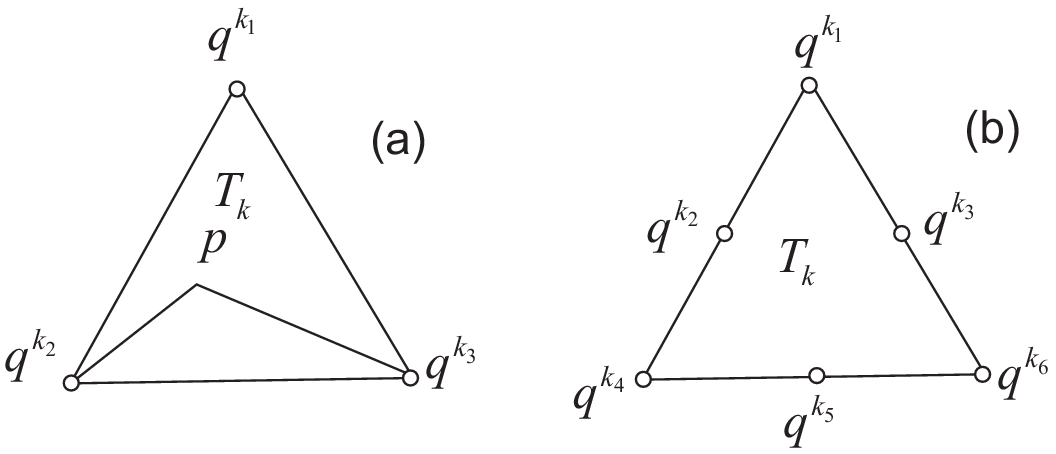Fig. 98 $$P_1$$ and $$P_2$$ degrees of freedom on triangle $$T_k$$

For each vertex $$q^i$$, the basis function $$\phi_i$$ in Vh(Th, P1) is given by:

$\begin{split}\phi_i(x,y)&=a^k_i+b^k_ix+c^k_iy \textrm{ for }(x,y)\in T_k,\\ \phi_i(q^i)&=1,\quad \phi_i(q^j)=0 \textrm{ if }i\neq j\end{split}$

The basis function $$\phi_{k_1}(x,y)$$ with the vertex $$q^{k_1}$$ in Fig. 98 at point $$p=(x,y)$$ in triangle $$T_k$$ simply coincide with the barycentric coordinates $$\lambda^k_1$$ (area coordinates):

$\phi_{k_1}(x,y) = \lambda^k_{1}(x,y)= \frac{\textrm{area of triangle} (p, q^{k_2},q^{k_3})} {\textrm{area of triangle}(q^{k_1},q^{k_2},q^{k_3})}$

If we write:

 1 2 Vh(Th, P1); Vh fh = g(x.y); 

then:

fh = $$\displaystyle f_h(x,y)=\sum_{i=1}^{n_v}f(q^i)\phi_i(x,y)$$

See Fig. 101 for the projection of $$f(x,y)=\sin(\pi x)\cos(\pi y)$$ into Vh(Th, P1).Fig. 99 Test mesh Th for projection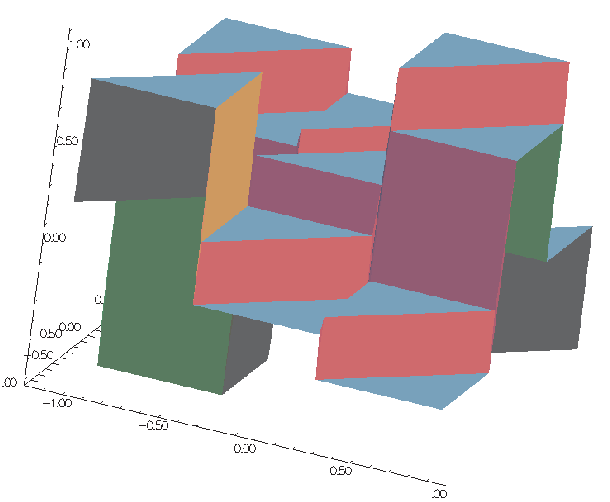Fig. 100 Projection to Vh(Th, P0)

Finite element P0

### P2-element

For each vertex or mid-point $$q^i$$. The basis function $$\phi_i$$ in Vh(Th, P2) is given by:

$\begin{split}\begin{array}{rcl} \phi_i(x,y)&=&a^k_i+b^k_ix+c^k_iy+d^k_ix^2+e^k_ixy+f^f_jy^2\textrm{ for }(x,y)\in T_k,\\ \phi_i(q^i)&=&1,\quad \phi_i(q^j)=0\textrm{ if }i\neq j \end{array}\end{split}$

The basis function $$\phi_{k_1}(x,y)$$ with the vertex $$q^{k_1}$$ in Fig. 98 is defined by the barycentric coordinates:

$\phi_{k_1}(x,y) = \lambda^k_{1}(x,y)(2\lambda^k_1(x,y)-1)$

and for the mid-point $$q^{k_2}$$:

$\phi_{k_2}(x,y) = 4\lambda^k_1(x,y)\lambda^k_4(x,y)$

If we write:

 1 2 Vh(Th, P2); Vh fh = f(x.y); 

then:

fh = $$\displaystyle f_h(x,y)=\sum_{i=1}^{M}f(q^i)\phi_i(x,y)\quad (\textrm{summation over all vertex or mid-point})$$

See Projection to Vh(Th, P2) for the projection of $$f(x,y)=\sin(\pi x)\cos(\pi y)$$ into Vh(Th, P2).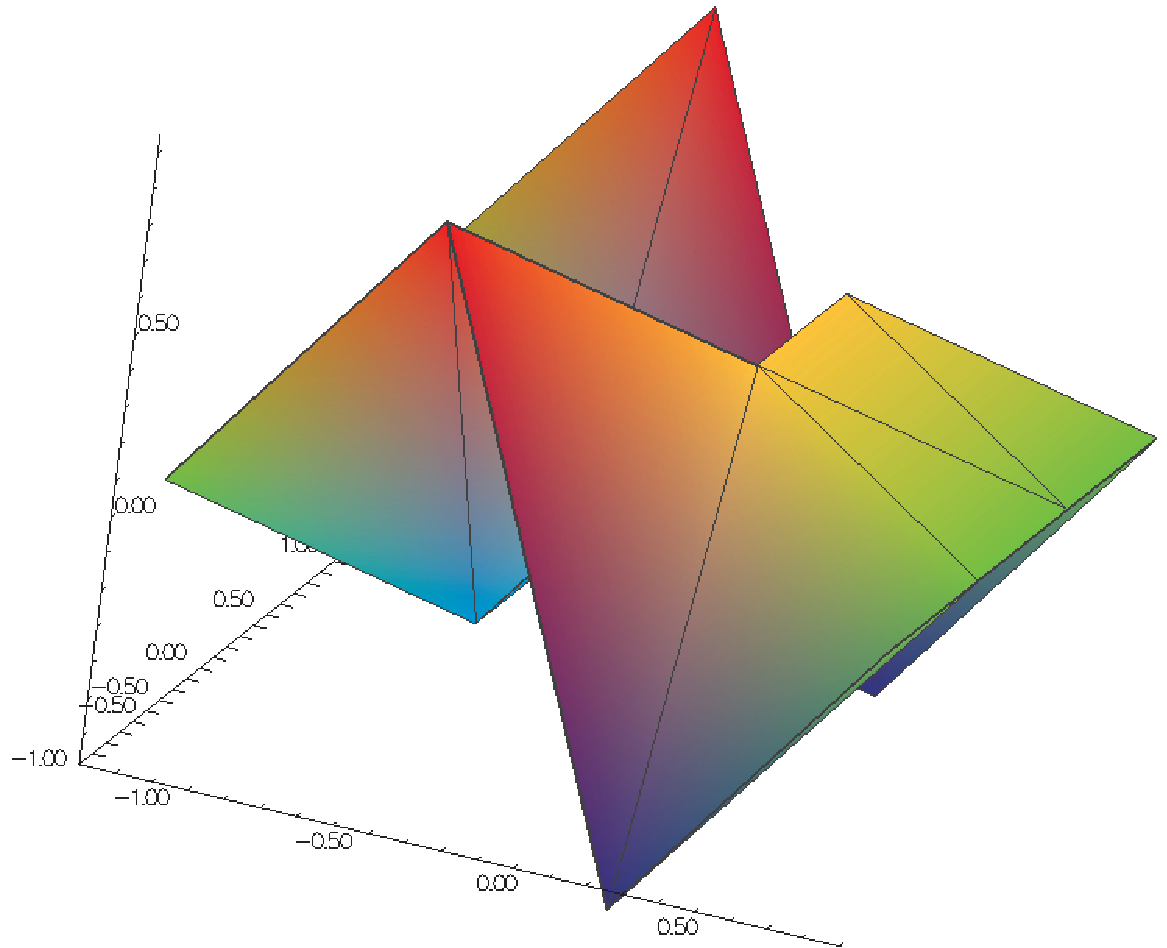Fig. 101 Projection to Vh(Th, P1)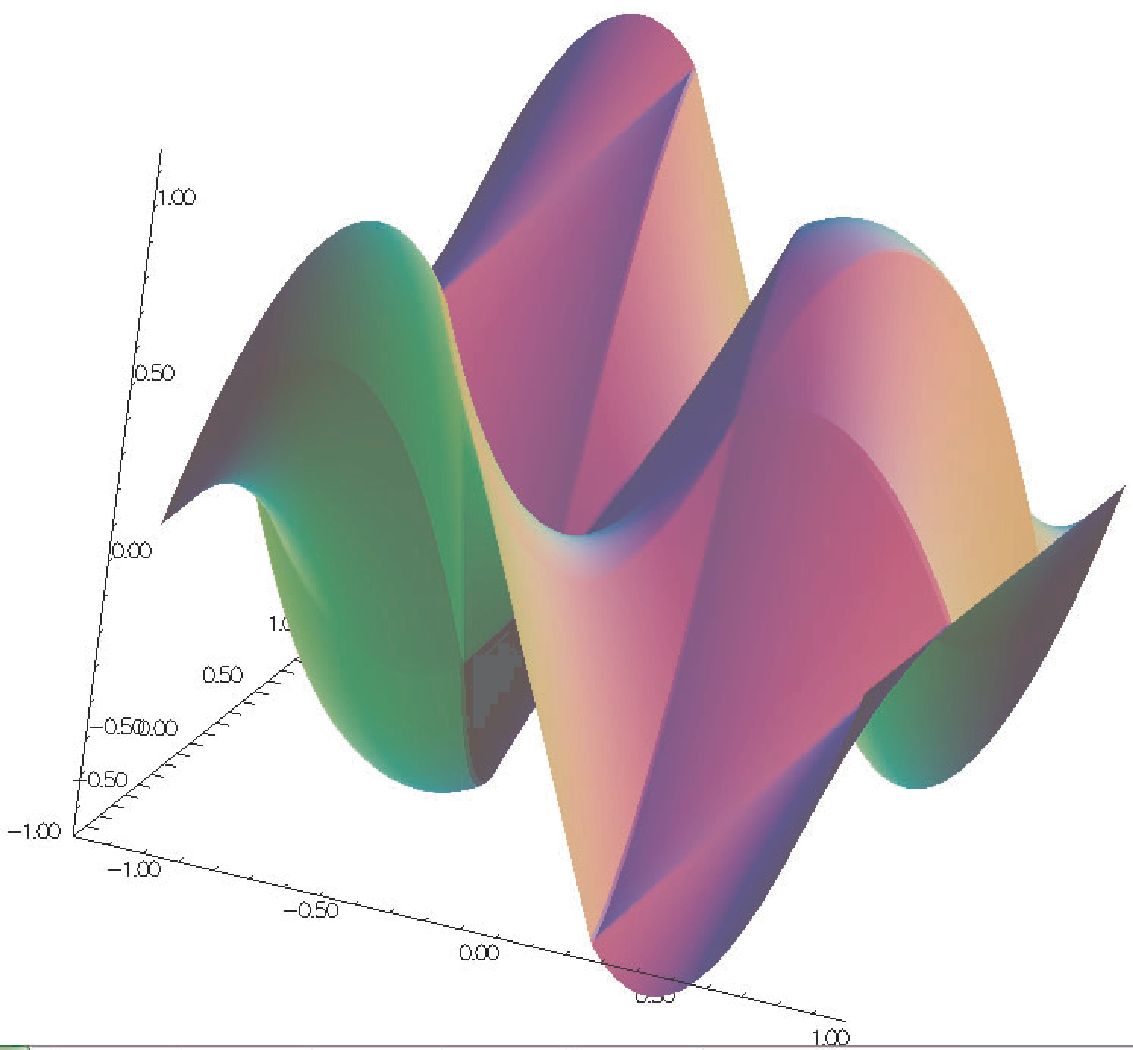Fig. 102 Projection to Vh(Th, P2)

Finite elements P1, P2

## Surface Lagrangian Finite Elements

### Definition of the surface P1 Lagragian element

To build the surface Pk-Lagrange, the main idea is to consider the usual 2d Lagrangian Finite Elements ; and its writing in barycentric coordinates ; apply a space transformation and barycentric properties. The FreeFEM finite elements for surface problem are: P0 P1 P2 P1b.

0) Notation

• Let $$\hat K$$ be the shape triangle in the space $$\mathbb{R}^2$$ of vertice $$(i_0,i_1,i_2)$$
• Let $$K$$ be a triangle of the space $$\mathbb{R}^3$$ of vertice $$(A_0,A_1,A_2)$$
• $$x_q$$ a quadrature point on K
• $$X_q$$ a quadrature point on A
• $$P1_{2d}$$ designates 2d P1 Lagrangian Finite Elements
• $$P1_{S}$$ designates surface 3d P1 Lagrangian Finite Elements
• $$(\lambda_i)_{i=0}^2$$ shape fonction of $$\hat K$$ ($$P1_{2d}$$)
• $$(\psi_i)_{i=0}^2$$ shape fonction of of $$K$$ ($$P1_S$$ )

1) Geometric transformation: from the current FE to the reference FE

Let be $$\hat x= \begin{pmatrix} \hat x \\ \hat y \end{pmatrix}$$ a point of the triangle $$\hat K \subset \mathbb{R}^2$$ and $$X= \begin{pmatrix} x \\ y \\ z \end{pmatrix}$$ a point of the triangle $$K \subset \mathbb{R}^3$$, where $$\hat x$$ and $$\hat X$$ are expressed in baricentric coordinates.

The motivation here is to parameterize the 3d surface mesh to the reference 2d triangle, thus to be reduced to a finite element 2d P1. Let’s define a geometric transformation F, such as $$F: \mathbb{R}^2 \rightarrow \mathbb{R}^3$$

However, thus defines transformation F as not bijective.

So, consider the following approximation

$\begin{split}\tilde F: \mathbb{R}^2 &\rightarrow \mathbb{R}^3 \\ \hat{x} &\rightarrow X \\ \begin{pmatrix} x \\ y \\ 0 \end{pmatrix} &\rightarrow \begin{pmatrix} \overrightarrow {A_0 A_1} \\ \overrightarrow {A_0A_2} \\ \overrightarrow {A_0A_1} \wedge \overrightarrow {A_0A_2} \end{pmatrix} ( \hat{x} -A_0)\end{split}$

where $$\wedge$$ denote the usual vector product.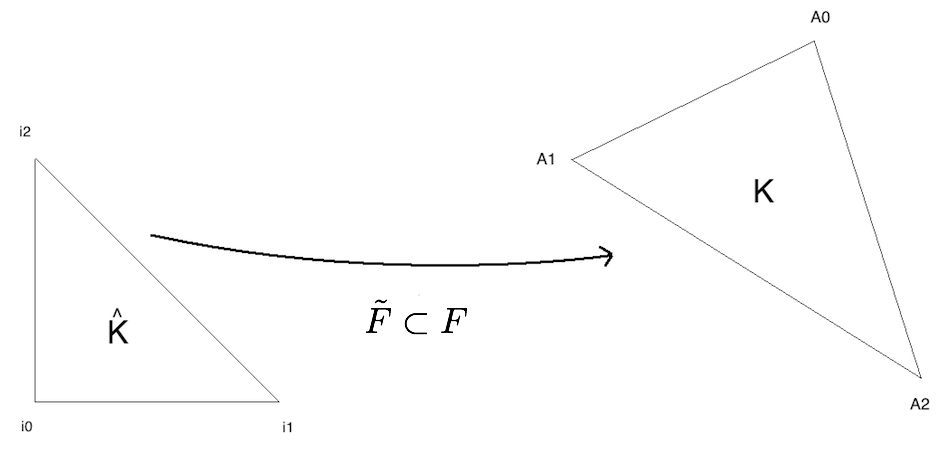Fig. 103 F, a parameterization from the reference 2d triangle to a 3d surface triangle

Note

$$\overrightarrow {A0A1} \wedge \overrightarrow {A_0A_2} = \begin{pmatrix} n_x \\ n_y \\ n_z \end{pmatrix}$$ defines the normal to the tangent plane generated by $$( A_0,\overrightarrow {A_0A_1}, \overrightarrow {A_0A_2} )$$

The affine transformation $$\tilde F$$ allows you to pass from the 2d reference triangle, which we project in $$\mathbb{R}^3$$ to the 3d current triangle, discretizing the surface we note $$\Gamma$$.

Then $$\tilde F^{-1}$$ is well defined and allows to return to the reference triangle $$\hat K$$, to the usual coordinates of $$\mathbb{R}^2$$ completed by the coordinate $$z=0$$.

2) Interpolation element fini

Remember that the reference geometric element for the finite element $$P1s$$ that we are building is the reference triangle $$\hat K$$ in the vertex plane $$(i_0, i_1, i_2$$), which we project into space by posing $$z=0$$ by the membrane hypothesis.

Hence $$i_0 = \begin{pmatrix} 0 \\ 0 \\ 0 \end{pmatrix}$$, $$i_1 = \begin{pmatrix} 1 \\ 0 \\ 0 \end{pmatrix}$$, $$i_1 = \begin{pmatrix} 0 \\ 1 \\ 0 \end{pmatrix}$$.

Let X be a point of the current triangle K, we have X= $$\tilde F(\hat{x})$$. The barycentric coordinates of X in K are given by: $$X = \sum_{i=0} ^2 A_i \hat \lambda(\hat{x})$$

• $$A_i$$ the points of the current triangle K
• $$\hat \lambda_i$$ basic functions $$P1_{2d}$$
• $$\hat \lambda_0(x,y) = 1-x-y$$
• $$\hat \lambda_1(x,y) = x$$
• $$\hat \lambda_2(x,y) = y$$

We need to define a quadrature formula for the finite element approximation. The usual formulation for a 2d triangle will be used by redefining the quadrature points $$X_q = x_q = \begin{pmatrix} \hat x_q \\ \hat y_q \\ 0 \end{pmatrix}$$.

3) The Lagragian P1 functions and theirs 1st order derivatives

The finite element interpolation gives us the following relationship: $$\psi_i(X) = F^{-1} (\psi_i)( F^{-1} (X))$$. To find the expression of the basic functions $$\psi$$ on the current triangle K, it is sufficient to use the inverse of the transformation $$\tilde F$$ to get back to the reference triangle $$\hat K$$. However in FreeFEM, the definition of the reference finite element, the current geometry is based on barycentric coordinates in order not to use geometric transformation. $$\tilde F$$. The method used here is geometric and based on the properties of the vector product and the area of the current triangle K.

i) The shape functions

Let be the triangle K of vertices $$i_0, i_1, i_2 \subset \mathbb{R}^3$$ and $$(\lambda_i)_{i=0} ^2$$ the local barycentric coordinates at K. The normal is defined as the tangent plane generated by $$(A_0, \overrightarrow {A_0A_1}, \overrightarrow {A_0A_2})$$, \ $$\vec n = \overrightarrow {A_0A_1} \wedge \overrightarrow {A_0A_2}$$ avec $$\mid \mid \vec n \mid \mid = 2 \text{ mes }(\hat K)$$.

Le denotes the operator V, defines the usual vector product of $$\mathbb{R}^3$$ such as $$V(A,B,C) = (B-A) \wedge (C-A)$$

The mixed product of three vectors u, v, w, noté $$[u, v, w]$$, is the determinant of these three vectors in any direct orthonormal basis, thus $$(A \wedge V,C)= \text{ det }(A,B,C)$$

with $$(.,.)$$ is the usual scalar product of $$\mathbb{R}^3$$. \ Let Ph :math: in mathbb{R}^3 and P his projected in the triangle K such as:

Let’s lay the sub-triangles as follows :

• $$K_0 = (P,A1,A2)$$
• $$K_1 = (A0,P,A2)$$
• $$K_2 = (A0,A1,P)$$

with $$K = K_0 \cup K_1 \cup K_2$$.

Note

Properties in $$\mathbb{R}^3$$
• Let $$\vec n$$ be the normal to the tangent plane generated by $$(A_0, \overrightarrow {A_0A_1}, \overrightarrow {A_0A_2})$$
• $$\vec n =\overrightarrow {A_0 A_1} \wedge \overrightarrow {A_0 A_2}$$
• By definition, $$\mathcal{A}= \frac{1}{2} \mid < \vec n,\vec n> \mid$$ and the vectorial area by $$\mathcal{A^S}= \frac{1}{2} < \vec n,\vec n>$$ hence $$\mathcal{A^S} (PBC)= \frac{1}{2} < \vec n_0,\vec n>$$, with $$\vec n_0$$ the normal vector to the plane (PBC)

Let’s define the respective vector areas

• $$\vec N_0(P) = V(P,A1,A2)$$ the vectorial area of K0
• $$\vec N_1(P) = V(A0,P,A2)$$ the vectorial area of K1
• $$\vec N_2(P) = V(A0,A1,P)$$ the vectorial area of K2

By definition, in 3d, the barycentric coordinates are given by algebraic area ratio: :math: lambda_i(P) = frac {(vec N_i(P),vec N)}{(vec N,vec N)}label{basisfunc}

Note that $$(\vec N_i(P) ,\vec N) = 2 \text{ sign } \text{ mes } (K_i) \mid \mid \vec N \mid \mid$$ and $$(\vec N,\vec N) = 2 \text{ sign } \text{ mes } (K) \mid \mid \vec N \mid \mid$$, avec $$sign$$ the orientation of the current triangle compared to the reference triangle.

We find the finite element interpolation, $$P = \sum_{i=0}^2 \lambda_i(P) A_i$$.

ii) 1st order derivatives of Lagrangian P1 FE

Let $$\vec Y$$ be any vector of $$\in \mathbb{R}^3$$.

\begin{split}\begin{aligned} (\vec N_2(P) ,\vec Y) &= ( (A_1-A_0) \wedge (P-A_0),Y) \\ &=\text{det}(A_1-A_0,P-A_0,Y) \\ &= \text{det}(A_1-A_0,P,Y)- \text{det}(A_1-A_0,A_0,Y) \end{aligned}\end{split}

Let’s calculate the differential of $$(\vec N_2(P),Y), \forall Y$$

\begin{split}D_P (\vec N_2(P) ,\vec Y) = \text{det} (A_1-A_0,P',Y) dP \\ \begin{aligned} \nabla_P (\vec N_2(P) ,\vec Y) &= \text{ det } (A_1-A_0, P' ,\vec Y) \\ &= - det (A_1-A_0, \vec Y, P') \\ &= - (A_1-A_0) \wedge \vec Y . P' \\ &= \vec Y \wedge (A_1-A_0) \end{aligned}\end{split}

Consider in particular $$\vec Y = \vec N$$, then

\begin{split}\begin{aligned} \nabla_P (\vec N_2(P) ,\vec N) &= \vec N \wedge (A_1-A_0) \\ &= \vec N \wedge E_2 &= - \text{det} (A_1-A_0, \vec Y, P') \end{aligned}\end{split}

This leads to :math: nabla_P lambda_2(P) = frac {(vec N wedge E_2)}{(vec N,vec N)}

By similar calculations for $$\vec N_0(P) et \vec N_1(P)$$

$$\nabla_P \lambda_i(P) = \frac {(\vec N \wedge E_i)}{(\vec N,\vec N)}\label{derivbasisfunc}$$

Note

With the definition of the surface gradient and the 2d Pk-Lagrange FE used barycentric coordinates, surface Pk-Langrange FE are trivial.

## P1 Nonconforming Element

Refer to [THOMASSET2012] for details; briefly, we now consider non-continuous approximations so we will lose the property:

$w_h\in V_h\subset H^1(\Omega)$

If we write:

 1 2 Vh(Th, P1nc); Vh fh = f(x.y); 

then:

fh = $$\displaystyle f_h(x,y)=\sum_{i=1}^{n_v}f(m^i)\phi_i(x,y)\quad (\textrm{summation over all midpoint})$$

Here the basis function $$\phi_i$$ associated with the mid-point $$m^i=(q^{k_i}+q^{k_{i+1}})/2$$ where $$q^{k_i}$$ is the $$i$$-th point in $$T_k$$, and we assume that $$j+1=0$$ if $$j=3$$:

$\begin{split}\phi_i(x,y) &= a^k_i+b^k_ix+c^k_iy~\textrm{for }(x,y)\in T_k,\\ \phi_i(m^i) &= 1,\quad \phi_i(m^j)=0\textrm{ if }i\neq j\end{split}$

Strictly speaking $$\p \phi_i/\p x,\, \p \phi_i/\p y$$ contain Dirac distribution $$\rho \delta_{\p T_k}$$.

The numerical calculations will automatically ignore them. In [THOMASSET2012], there is a proof of the estimation

$\left(\sum_{k=1}^{n_v}\int_{T_k}|\nabla w-\nabla w_h|^2\d x\d y\right)^{1/2} =O(h)$

The basis functions $$\phi_k$$ have the following properties.

1. For the bilinear form $$a$$ defined in Fig. 104 satisfy:

$\begin{split}\begin{array}{rcl} a(\phi_i,\phi_i)>0,\qquad a(\phi_i,\phi_j)&\le& 0\quad\textrm{if }i\neq j\\ \sum_{k=1}^{n_v}a(\phi_i,\phi_k)&\ge& 0 \end{array}\end{split}$
2. $$f\ge 0 \Rightarrow u_h\ge 0$$

3. If $$i\neq j$$, the basis function $$\phi_i$$ and $$\phi_j$$ are $$L^2$$-orthogonal:

$\int_{\Omega}\phi_i\phi_j\, \d x\d y=0\qquad \textrm{if }i\neq j$

which is false for $$P_1$$-element.

See Fig. 104 for the projection of $$f(x,y)=\sin(\pi x)\cos(\pi y)$$ into Vh(Th, P1nc).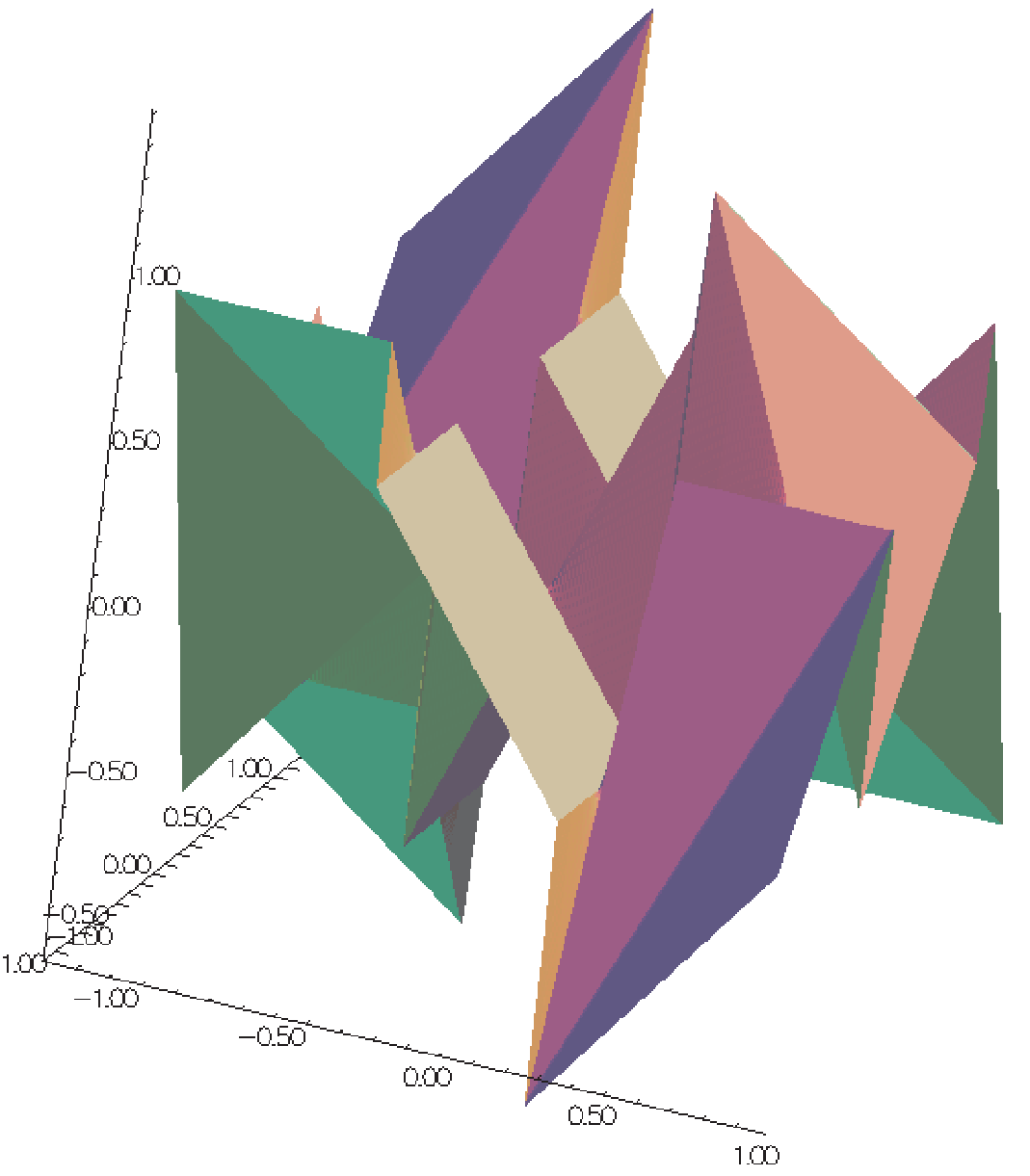Fig. 104 Projection to Vh(Th, P1nc)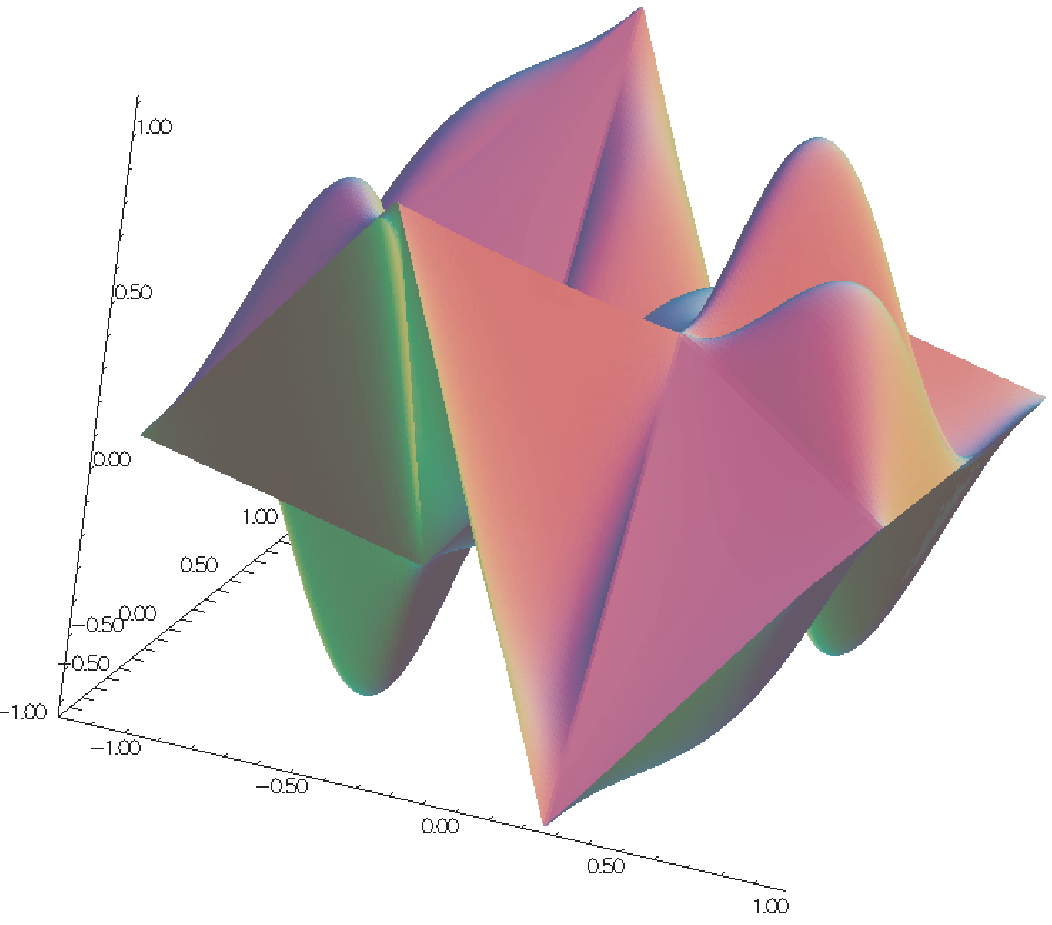Fig. 105 Projection to Vh(Th, P1b)

Finite elements P1nc, P1b

## Other FE-space

For each triangle $$T_k\in \mathcal{T}_h$$, let $$\lambda_{k_1}(x,y),\, \lambda_{k_2}(x,y),\, \lambda_{k_3}(x,y)$$ be the area cordinate of the triangle (see Fig. 98), and put:

$\beta_k(x,y)=27\lambda_{k_1}(x,y)\lambda_{k_2}(x,y)\lambda_{k_3}(x,y)$

called bubble function on $$T_k$$. The bubble function has the feature: 1. $$\beta_k(x,y)=0\quad \textrm{if }(x,y)\in \p T_k$$.

1. $$\beta_k(q^{k_b})=1$$ where $$q^{k_b}$$ is the barycenter $$\frac{q^{k_1}+q^{k_2}+q^{k_3}}{3}$$.

If we write:

 1 2 Vh(Th, P1b); Vh fh = f(x.y); 

then:

fh = $$\displaystyle f_h(x,y)=\sum_{i=1}^{n_v}f(q^i)\phi_i(x,y)+\sum_{k=1}^{n_t}f(q^{k_b})\beta_k(x,y)$$

See Fig. 105 for the projection of $$f(x,y)=\sin(\pi x)\cos(\pi y)$$ into Vh(Th, P1b).

## Vector Valued FE-function

Functions from $$\R^{2}$$ to $$\R^{N}$$ with $$N=1$$ are called scalar functions and called vector valued when $$N>1$$. When $$N=2$$

 1 fespace Vh(Th, [P0, P1]) ; 

makes the space

$V_h=\{\mathbf{w}=(w_1,w_2)|\; w_1\in V_h(\mathcal{T}_h,P_0),\, w_2\in V_h(\mathcal{T}_h,P_1)\}$

### Raviart-Thomas Element

In the Raviart-Thomas finite element $$RT0_{h}$$, the degrees of freedom are the fluxes across edges $$e$$ of the mesh, where the flux of the function $$\mathbf{f} : \R^2 \longrightarrow \R^2$$ is $$\int_{e} \mathbf{f}.n_{e}$$, $$n_{e}$$ is the unit normal of edge $$e$$.

This implies an orientation of all the edges of the mesh, for example we can use the global numbering of the edge vertices and we just go from small to large numbers.

To compute the flux, we use a quadrature with one Gauss point, the mid-point of the edge.

Consider a triangle $$T_k$$ with three vertices $$(\mathbf{a},\mathbf{b},\mathbf{c})$$.

Lets denote the vertices numbers by $$i_{a},i_{b},i_{c}$$, and define the three edge vectors $$\mathbf{e}^{1},\mathbf{e}^{2},\mathbf{e}^{3}$$ by $$sgn(i_{b}-i_{c})(\mathbf{b}-\mathbf{c})$$, $$sgn(i_{c}-i_{a})(\mathbf{c}-\mathbf{a})$$, $$sgn(i_{a}-i_{b})(\mathbf{a}-\mathbf{b})$$.

We get three basis functions:

$\boldsymbol{\phi}^{k}_{1}= \frac{sgn(i_{b}-i_{c})}{2|T_k|}(\mathbf{x}-\mathbf{a}),\quad \boldsymbol{\phi}^{k}_{2}= \frac{sgn(i_{c}-i_{a})}{2|T_k|}(\mathbf{x}-\mathbf{b}),\quad \boldsymbol{\phi}^{k}_{3}= \frac{sgn(i_{a}-i_{b})}{2|T_k|}(\mathbf{x}-\mathbf{c}),$

where $$|T_k|$$ is the area of the triangle $$T_k$$. If we write:

 1 2 Vh(Th, RT0); Vh [f1h, f2h] = [f1(x, y), f2(x, y)]; 

then:

fh = $$\displaystyle \mathbf{f}_h(x,y)=\sum_{k=1}^{n_t}\sum_{l=1}^6 n_{i_lj_l}|\mathbf{e^{i_l}}|f_{j_l}(m^{i_l})\phi_{i_lj_l}$$

where $$n_{i_lj_l}$$ is the $$j_l$$-th component of the normal vector $$\mathbf{n}_{i_l}$$,

$\{m_1,m_2,m_3\} = \left\{\frac{\mathbf{b}+\mathbf{c}}{2}, \frac{\mathbf{a}+\mathbf{c}}{2}, \frac{\mathbf{b}+\mathbf{a}}{2} \right\}$

and $$i_l=\{1,1,2,2,3,3\},\, j_l=\{1,2,1,2,1,2\}$$ with the order of $$l$$.

  1 2 3 4 5 6 7 8 9 10 11 12 13 14 15 16 17 18 19 20 21 22 23 24 25 26 27 // Mesh mesh Th = square(2, 2); // Fespace fespace Xh(Th, P1); Xh uh = x^2 + y^2, vh; fespace Vh(Th, RT0); Vh [Uxh, Uyh] = [sin(x), cos(y)]; //vectorial FE function // Change the mesh Th = square(5,5); //Xh is unchanged //Uxh = x; //error: impossible to set only 1 component //of a vector FE function vh = Uxh;//ok //and now vh use the 5x5 mesh //but the fespace of vh is always the 2x2 mesh // Plot plot(uh); uh = uh; //do a interpolation of uh (old) of 5x5 mesh //to get the new uh on 10x10 mesh plot(uh); vh([x-1/2, y]) = x^2 + y^2; //interpolate vh = ((x-1/2)^2 + y^2)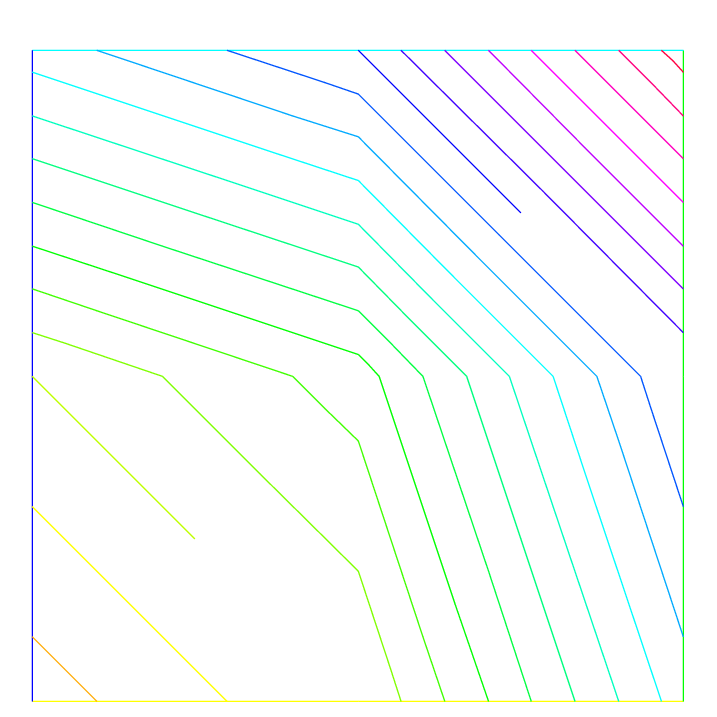Fig. 107 vh Iso on mesh $$2\times 2$$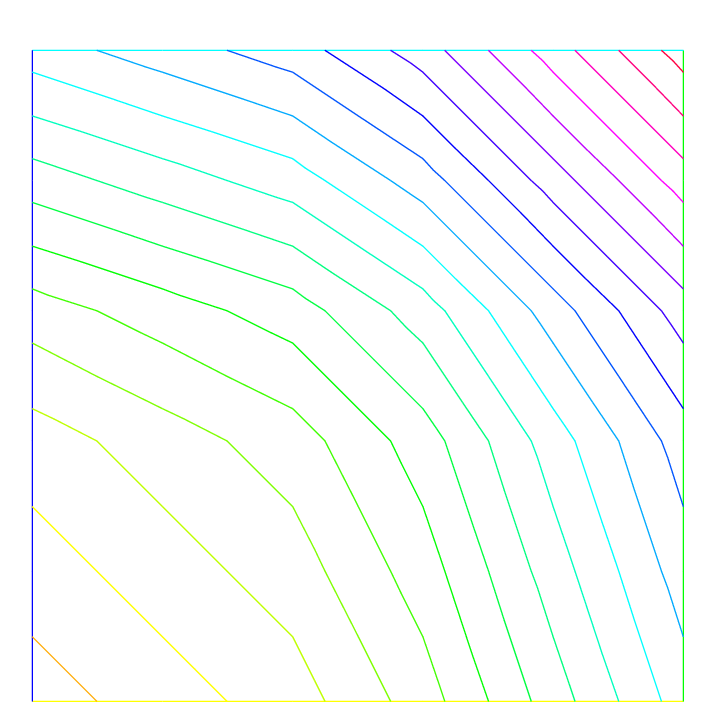Fig. 108 vh Iso on mesh $$5\times 5$$

To get the value at a point $$x=1,y=2$$ of the FE function uh, or [Uxh, Uyh], one writes:

 1 2 3 4 5 6 7 8 real value; value = uh(2,4); //get value = uh(2, 4) value = Uxh(2, 4); //get value = Uxh(2, 4) //OR x = 1; y = 2; value = uh; //get value = uh(1, 2) value = Uxh; //get value = Uxh(1, 2) value = Uyh; //get value = Uyh(1, 2) 

To get the value of the array associated to the FE function uh, one writes

 1 2 3 4 real value = uh[]; //get the value of degree of freedom 0 real maxdf = uh[].max; //maximum value of degree of freedom int size = uh.n; //the number of degree of freedom real[int] array(uh.n) = uh[]; //copy the array of the function uh 

Warning

For a non-scalar finite element function [Uxh, Uyh] the two arrays Uxh[] and Uyh[] are the same array, because the degree of freedom can touch more than one component.

## A Fast Finite Element Interpolator

In practice, one may discretize the variational equations by the Finite Element method. Then there will be one mesh for $$\Omega_1$$ and another one for $$\Omega_2$$. The computation of integrals of products of functions defined on different meshes is difficult.

Quadrature formula and interpolations from one mesh to another at quadrature points are needed. We present below the interpolation operator which we have used and which is new, to the best of our knowledge.

Let $${\cal T}_{h}^0=\cup_k T^0_k,{\cal T}_{h}^1=\cup_k T^1_k$$ be two triangulations of a domain $$\Omega$$. Let:

$V({\hbox{{\cal T}}_{h}^i}) =\{ C^0(\Omega_h^i)~:~f|_{T^i_k}\in P_0\},~~~i=0,1$

be the spaces of continuous piecewise affine functions on each triangulation.

Let $$f\in V({\cal T}_{h}^0)$$. The problem is to find $$g\in V({\cal T}_{h}^1)$$ such that:

$g(q) = f(q) \quad \forall q\hbox{~vertex of ~} {\cal T}_{h}^1$

Although this is a seemingly simple problem, it is difficult to find an efficient algorithm in practice.

We propose an algorithm which is of complexity $$N^1\log N^0$$, where $$N^i$$ is the number of vertices of $$\cal T_{h}^i$$, and which is very fast for most practical 2D applications.

Algorithm

The method has 5 steps.

First a quadtree is built containing all the vertices of the mesh $${\cal T}_{h}^0$$ such that in each terminal cell there are at least one, and at most 4, vertices of $${\cal T}_{h}^0$$.

For each $$q^1$$, vertex of $${\cal T}_{h}^1$$ do:

1. Find the terminal cell of the quadtree containing $$q^1$$.

2. Find the the nearest vertex $$q^0_j$$ to $$q^1$$ in that cell.

3. Choose one triangle $$T_k^0\in{\cal T}_{h}^0$$ which has $$q^0_j$$ for vertex.

4. Compute the barycentric coordinates $$\{\lambda_j\}_{j=1,2,3}$$ of $$q^1$$ in $$T^0_k$$.

• if all barycentric coordinates are positive, go to Step 5
• otherwise, if one barycentric coordinate $$\lambda_i$$ is negative, replace $$T^0_k$$ by the adjacent triangle opposite $$q^0_i$$ and go to Step 4.
• otherwise, if two barycentric coordinates are negative, take one of the two randomly and replace $$T^0_k$$ by the adjacent triangle as above.
5. Calculate $$g(q^1)$$ on $$T^0_k$$ by linear interpolation of $$f$$:

$g(q^1) = \sum_{j=1,2,3} \lambda_j f(q^0_j)$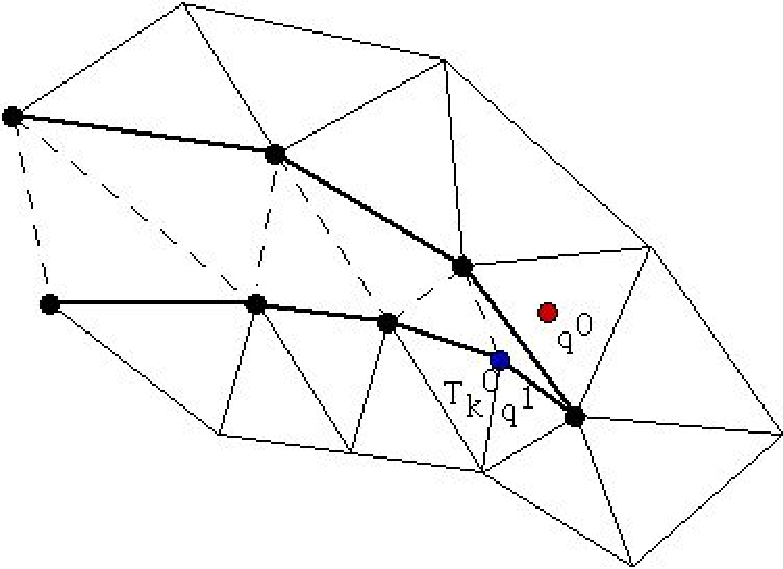Fig. 109 To interpolate a function at $$q^0$$, the knowledge of the triangle which contains $$q^0$$ is needed. The algorithm may start at $$q^1\in T_k^0$$ and stall on the boundary (thick line) because the line $$q^0q^1$$ is not inside $$\Omega$$. But if the holes are triangulated too (doted line) then the problem does not arise.

Two problems need to be solved:

• What if :math:q^1 is not in $$\Omega^0_h$$ ? Then Step 5 will stop with a boundary triangle.

So we add a step which tests the distance of $$q^1$$ with the two adjacent boundary edges and selects the nearest, and so on till the distance grows.

• What if $$\Omega^0_h$$ is not convex and the marching process of Step 4 locks on a boundary? By construction Delaunay-Voronoï’s mesh generators always triangulate the convex hull of the vertices of the domain.

Therefore, we make sure that this information is not lost when $${\cal T}_{h}^0,{\cal T}_{h}^1$$ are constructed and we keep the triangles which are outside the domain on a special list.

That way, in step 5 we can use that list to step over holes if needed.

Note

Sometimes, in rare cases, the interpolation process misses some points, we can change the search algorithm through a global variable searchMethod

 1 2 3 4 searchMethod = 0; // default value for fast search algorithm searchMethod = 1; // safe search algorithm, uses brute force in case of missing point // (warning: can be very expensive in cases where a lot of points are outside of the domain) searchMethod = 2; // always uses brute force. It is very computationally expensive. 

Note

Step 3 requires an array of pointers such that each vertex points to one triangle of the triangulation.

Note

The operator = is the interpolation operator of FreeFEM, the continuous finite functions are extended by continuity to the outside of the domain.

Try the following example :

  1 2 3 4 5 6 7 8 9 10 11 12 13 14 15 16 17 18 // Mesh mesh Ths = square(10, 10); mesh Thg = square(30, 30, [x*3-1, y*3-1]); plot(Ths, Thg, wait=true); // Fespace fespace Ch(Ths, P2); Ch us = (x-0.5)*(y-0.5); fespace Dh(Ths, P2dc); Dh vs = (x-0.5)*(y-0.5); fespace Fh(Thg, P2dc); Fh ug=us, vg=vs; // Plot plot(us, ug, wait=true); plot(vs, vg, wait=true);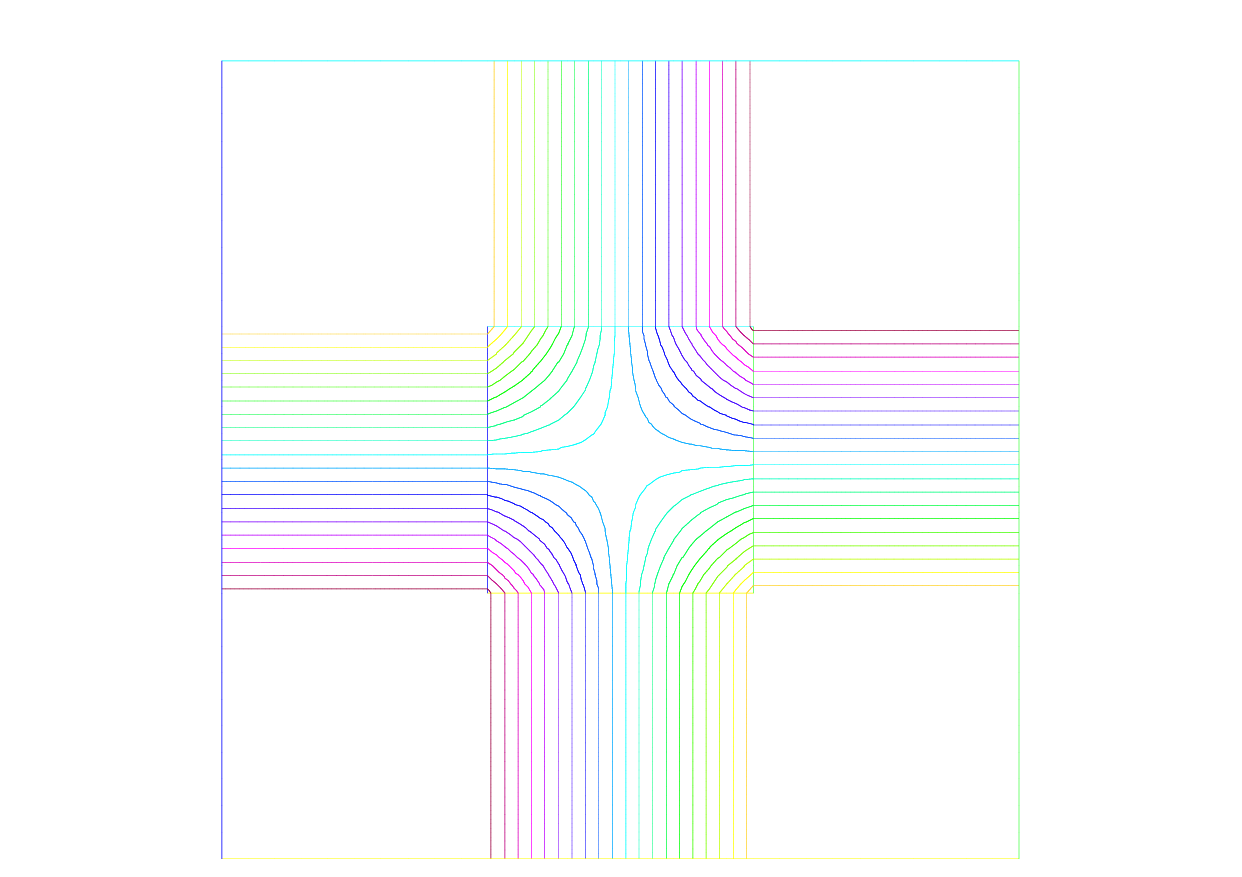Fig. 110 Extension of a continuous FE-function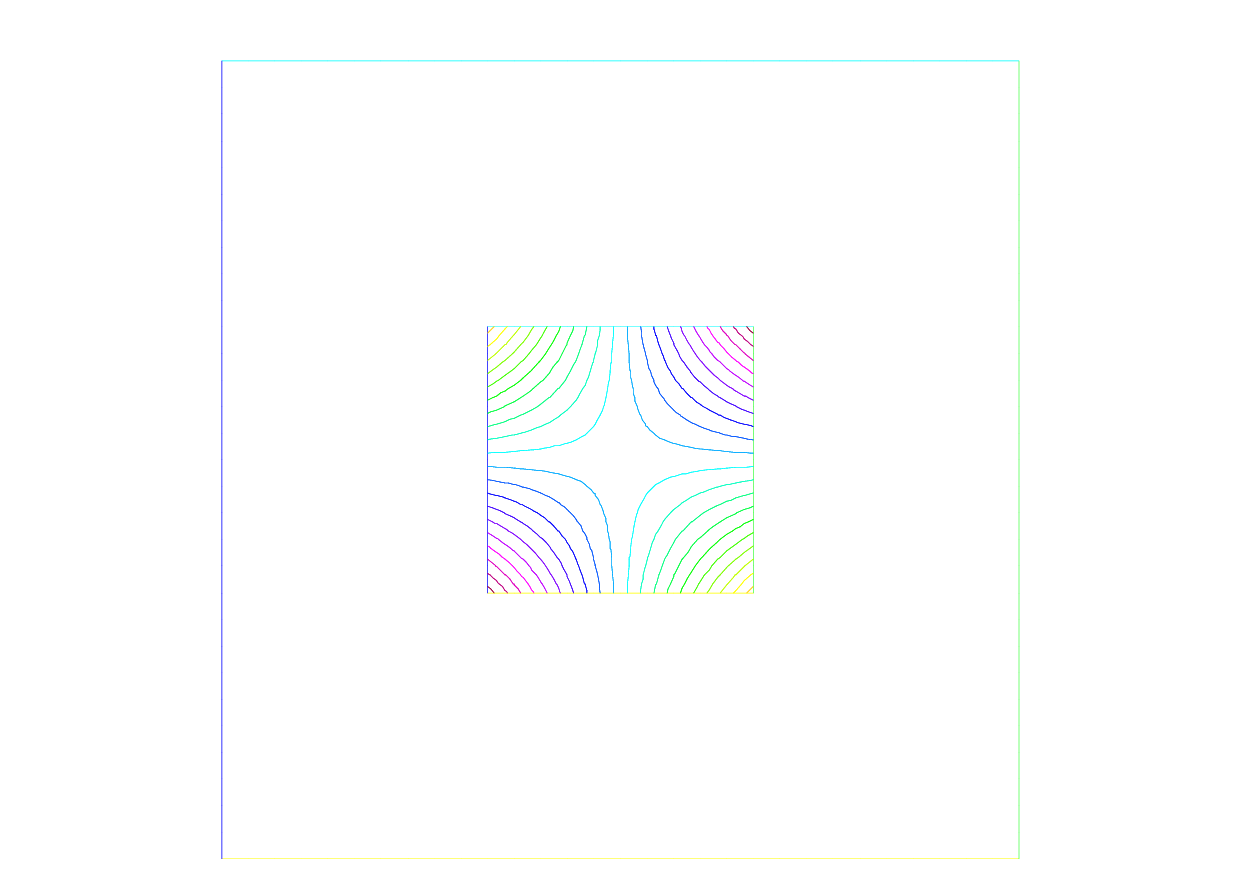Fig. 111 Extension of discontinuous FE-function

Extension of FE-function

## Keywords: Problem and Solve

For FreeFEM, a problem must be given in variational form, so we need a bilinear form $$a(u,v)$$, a linear form $$\ell(f,v)$$, and possibly a boundary condition form must be added.

 1 2 3 4 problem P (u, v) = a(u,v) - l(f,v) + (boundary condition) ; 

Note

When you want to formulate the problem and solve it in the same time, you can use the keyword solve.

### Weak Form and Boundary Condition

To present the principles of Variational Formulations, also called weak form, for the Partial Differential Equations, let’s take a model problem: a Poisson equation with Dirichlet and Robin Boundary condition.

The problem: Find $$u$$ a real function defined on a domain $$\Omega$$ of $$\R^d$$ $$(d=2,3)$$ such that:

$\begin{split}\begin{array}{rcll} -\nabla\cdot(\kappa \nabla u) &=& f & \mbox{ in }\Omega\\ a u + \kappa \frac{\p u}{\p n} &=& b & \mbox{ on }\Gamma_r\\ u &=& g & \mbox{ on }\Gamma_d \end{array}\end{split}$

where:

• if $$d=2$$ then $$\nabla.(\kappa \nabla u) = \p_x(\kappa \p_x u ) + \p_y(\kappa \p_y u )$$ with $$\p_x u = \frac{\p u}{\p x}$$ and $$\p_y u = \frac{\p u}{\p y}$$
• if $$d=3$$ then $$\nabla.(\kappa \nabla u) = \p_x(\kappa \p_x u) + \p_y(\kappa \p_y u) + \p_z(\kappa \p_z u)$$ with $$\p_x u = \frac{\p u}{\p x}$$, $$\p_y u = \frac{\p u}{\p y}$$ and , $$\p_z u = \frac{\p u}{\p z}$$
• The border $$\Gamma=\p \Omega$$ is split in $$\Gamma_d$$ and $$\Gamma_n$$ such that $$\Gamma_d \cap \Gamma_n = \emptyset$$ and $$\Gamma_d \cup \Gamma_n = \p \Omega$$,
• $$\kappa$$ is a given positive function, such that $$\exists \kappa_0 \in \R ,\quad 0 < \kappa_0 \leq \kappa$$.
• $$a$$ a given non negative function,
• $$b$$ a given function.

Note

This is the well known Neumann boundary condition if $$a=0$$, and if $$\Gamma_d$$ is empty.

In this case the function appears in the problem just by its derivatives, so it is defined only up to a constant (if $$u$$ is a solution then $$u+c$$ is also a solution).

Let $${v}$$, a regular test function, null on $$\Gamma_d$$, by integration by parts we get:

$- \int_{\Omega} \nabla\cdot(\kappa \nabla u) \, {v} \,d\omega = \int_{\Omega} \kappa \nabla{ v} \cdot \nabla u \,d\omega - \int_{\Gamma} {v}\kappa \frac{ \p u}{\p \mathbf{n}} \,d\gamma,= \int_{\Omega} f {v} \,d\omega$

where if $$d=2$$ the $$\nabla{ v} . \nabla u = (\frac{\p u}{\p x}\frac{\p { v}}{\p x}+\frac{\p u}{\p y}\frac{\p { v}}{\p y})$$,

where if $$d=3$$ the $$\nabla{ v} . \nabla u = (\frac{\p u}{\p x}\frac{\p { v}}{\p x}+\frac{\p u}{\p y}\frac{\p { v}}{\p y} + \frac{\p u}{\p z}\frac{\p { v}}{\p z})$$,

and where $$\mathbf{n}$$ is the unitary outer-pointing normal of the $$\Gamma$$.

Now we note that $$\kappa \frac{ \p u}{\p n} = - a u + b$$ on $$\Gamma_r$$ and $$v=0$$ on $$\Gamma_d$$ and $$\Gamma = \Gamma_d \cup \Gamma_n$$ thus:

$- \int_{\Gamma} {v} \kappa \frac{ \p u}{\p n} = \int_{\Gamma_r} a u v - \int_{\Gamma_r} b v$

The problem becomes:

Find $$u \in V_g = \{w \in H^1(\Omega) / w = g \mbox{ on } \Gamma_d \}$$ such that:

(23) ${\int_{\Omega} \kappa \nabla{ v} . \nabla u \,d\omega + \int_{\Gamma_r} a u v \,d\gamma = \int_{\Omega} f {v}} \,d\omega + \int_{\Gamma_r} b v \,d\gamma , \quad \forall v \in V_0$

where $$V_0 = \{v \in H^1(\Omega) / v = 0 \mbox{ on } \Gamma_d \}$$

Except in the case of Neumann conditions everywhere, the problem (23) is well posed when $$\kappa\geq \kappa_0>0$$.

Note

If we have only the Neumann boundary condition, linear algebra tells us that the right hand side must be orthogonal to the kernel of the operator for the solution to exist.

One way of writing the compatibility condition is:

$\int_{\Omega} f \,d\omega + \int_{\Gamma} b \,d\gamma=0$

and a way to fix the constant is to solve for $$u \in H^1(\Omega)$$ such that:

${\int_{\Omega} (\varepsilon u v \; + \; \kappa \nabla{ v} . \nabla u) \,d\omega = \int_{\Omega} f {v}} \,d\omega + \int_{\Gamma_r} b v \,d\gamma , \quad \forall v \in H^1(\Omega)$

where $$\varepsilon$$ is a small parameter ($$\sim \kappa\; 10^{-10} |\Omega|^{\frac2d}$$).

Remark that if the solution is of order $$\frac{1}{\varepsilon}$$ then the compatibility condition is unsatisfied, otherwise we get the solution such that $$\int_\Omega u = 0$$, you can also add a Lagrange multiplier to solve the real mathematical problem like in the Lagrange multipliers example.

In FreeFEM, the bidimensional problem (23) becomes:

  1 2 3 4 5 6 7 8 9 10 11 12 13 14 15 problem Pw (u, v) = int2d(Th)( //int_{Omega} kappa nabla v . nabla u kappa*(dx(u)*dx(v) + dy(u)*dy(v)) ) + int1d(Th, gn)( //int_{Gamma_r} a u v a * u*v ) - int2d(Th)( //int_{Omega} f v f*v ) - int1d(Th, gn)( //int_{Gamma_r} b v b * v ) + on(gd, u=g) //u = g on Gamma_d ; 

where Th is a mesh of the bi-dimensional domain $$\Omega$$, and gd and gn are respectively the boundary labels of boundary $$\Gamma_d$$ and $$\Gamma_n$$.

And the three dimensional problem (23) becomes

  1 2 3 4 5 6 7 8 9 10 11 12 13 14 15 16 macro Grad(u) [dx(u), dy(u), dz(u) ]// problem Pw (u, v) = int3d(Th)( //int_{Omega} kappa nabla v . nabla u kappa*(Grad(u)'*Grad(v)) ) + int2d(Th, gn)( //int_{Gamma_r} a u v a * u*v ) - int3d(Th)( //int_{Omega} f v f*v ) - int2d(Th, gn)( //int_{Gamma_r} b v b * v ) + on(gd, u=g) //u = g on Gamma_d ; 

where Th is a mesh of the three dimensional domain $$\Omega$$, and gd and gn are respectively the boundary labels of boundary $$\Gamma_d$$ and $$\Gamma_n$$.

## Parameters affecting solve and problem

The parameters are FE functions real or complex, the number $$n$$ of parameters is even ($$n=2*k$$), the $$k$$ first function parameters are unknown, and the $$k$$ last are test functions.

Note

If the functions are a part of vectorial FE then you must give all the functions of the vectorial FE in the same order (see Poisson problem with mixed finite element for example).

Note

Don’t mix complex and real parameters FE function.

Warning

Bug:

The mixing of multiple fespace with different periodic boundary conditions are not implemented.

So all the finite element spaces used for tests or unknown functions in a problem, must have the same type of periodic boundary conditions or no periodic boundary conditions.

No clean message is given and the result is unpredictable.

The parameters are:

• solver= LU, CG, Crout, Cholesky, GMRES, sparsesolver, UMFPACK

The default solver is sparsesolver (it is equal to UMFPACK if no other sparse solver is defined) or is set to LU if no direct sparse solver is available.

The storage mode of the matrix of the underlying linear system depends on the type of solver chosen; for LU the matrix is sky-line non symmetric, for Crout the matrix is sky-line symmetric, for Cholesky the matrix is sky-line symmetric positive definite, for CG the matrix is sparse symmetric positive, and for GMRES, sparsesolver or UMFPACK the matrix is just sparse.

• eps= a real expression.

$$\varepsilon$$ sets the stopping test for the iterative methods like CG.

Note that if $$\varepsilon$$ is negative then the stopping test is:

$|| A x - b || < |\varepsilon|$

if it is positive, then the stopping test is:

$|| A x - b || < \frac{|\varepsilon|}{|| A x_{0} - b ||}$
• init= boolean expression, if it is false or 0 the matrix is reconstructed.

Note that if the mesh changes the matrix is reconstructed too.

• precon= name of a function (for example P) to set the preconditioner.

The prototype for the function P must be:

 1 func real[int] P(real[int] & xx); 
• tgv= Huge value ($$10^{30}$$) used to implement Dirichlet boundary conditions.

• tolpivot= sets the tolerance of the pivot in UMFPACK ($$10^{-1}$$) and, LU, Crout, Cholesky factorisation ($$10^{-20}$$).

• tolpivotsym= sets the tolerance of the pivot sym in UMFPACK

• strategy= sets the integer UMFPACK strategy ($$0$$ by default).

## Problem definition

Below v is the unknown function and w is the test function.

After the “=” sign, one may find sums of:

• Identifier(s); this is the name given earlier to the variational form(s) (type varf ) for possible reuse.

Remark, that the name in the varf of the unknown test function is forgotten, we use the order in the argument list to recall names as in a C++ function,

• The terms of the bilinear form itself: if $$K$$ is a given function,

• Bilinear part for 3D meshes Th

• int3d(Th)(K*v*w) = $$\displaystyle\sum_{T\in\mathtt{Th}}\int_{T } K\,v\,w$$
• int3d(Th, 1)(K*v*w) = $$\displaystyle\sum_{T\in\mathtt{Th},T\subset \Omega_{1}}\int_{T} K\,v\,w$$
• int3d(Th, levelset=phi)(K*v*w) = $$\displaystyle\sum_{T\in\mathtt{Th}}\int_{T,\phi<0} K\,v\,w$$
• int3d(Th, l, levelset=phi)(K*v*w) = $$\displaystyle\sum_{T\in\mathtt{Th},T\subset \Omega_{l}}\int_{T,\phi<0} K\,v\,w$$
• int2d(Th, 2, 5)(K*v*w) = $$\displaystyle\sum_{T\in\mathtt{Th}}\int_{(\p T\cup\Gamma) \cap ( \Gamma_2 \cup \Gamma_{5})} K\,v\,w$$
• int2d(Th, 1)(K*v*w) = $$\displaystyle\sum_{T\in\mathtt{Th},T\subset \Omega_{1}}\int_{T} K\,v\,w$$
• int2d(Th, 2, 5)(K*v*w) = $$\displaystyle\sum_{T\in\mathtt{Th}}\int_{(\p T\cup\Gamma) \cap (\Gamma_2 \cup \Gamma_{5})} K\,v\,w$$
• int2d(Th, levelset=phi)(K*v*w) = $$\displaystyle\sum_{T\in\mathtt{Th}}\int_{T,\phi=0} K\,v\,w$$
• int2d(Th, l, levelset=phi)(K*v*w) = $$\displaystyle\sum_{T\in\mathtt{Th},T\subset \Omega_{l}}\int_{T,\phi=0} K\,v\,w$$
• intallfaces(Th)(K*v*w) = $$\displaystyle\sum_{T\in\mathtt{Th}}\int_{\p T } K\,v\,w$$
• intallfaces(Th, 1)(K*v*w) = $$\displaystyle\sum_{{T\in\mathtt{Th},T\subset \Omega_{1}}}\int_{\p T } K\,v\,w$$
• They contribute to the sparse matrix of type matrix which, whether declared explicitly or not, is constructed by FreeFEM.
• Bilinear part for 2D meshes Th

• int2d(Th)(K*v*w) = $$\displaystyle\sum_{T\in\mathtt{Th}}\int_{T } K\,v\,w$$
• int2d(Th, 1)(K*v*w) = $$\displaystyle\sum_{T\in\mathtt{Th},T\subset \Omega_{1}}\int_{T} K\,v\,w$$
• int2d(Th, levelset=phi)(K*v*w) = $$\displaystyle\sum_{T\in\mathtt{Th}}\int_{T,\phi<0} K\,v\,w$$
• int2d(Th, l, levelset=phi)(K*v*w) = $$\displaystyle\sum_{T\in\mathtt{Th},T\subset \Omega_{l}}\int_{T,\phi<0} K\,v\,w$$
• int1d(Th, 2, 5)(K*v*w) = $$\displaystyle\sum_{T\in\mathtt{Th}}\int_{(\p T\cup\Gamma) \cap ( \Gamma_2 \cup \Gamma_{5})} K\,v\,w$$
• int1d(Th, 1)(K*v*w) = $$\displaystyle\sum_{T\in\mathtt{Th},T\subset \Omega_{1}}\int_{T} K\,v\,w$$
• int1d(Th, 2, 5)(K*v*w) = $$\displaystyle\sum_{T\in\mathtt{Th}}\int_{(\p T\cup\Gamma) \cap ( \Gamma_2 \cup \Gamma_{5})} K\,v\,w$$
• int1d(Th, levelset=phi)(K*v*w) = $$\displaystyle\sum_{T\in\mathtt{Th}}\int_{T,\phi=0} K\,v\,w$$
• int1d(Th, l, levelset=phi)(K*v*w) = $$\displaystyle\sum_{T\in\mathtt{Th},T\subset \Omega_{l}}\int_{T,\phi=0} K\,v\,w$$
• intalledges(Th)(K*v*w) = $$\displaystyle\sum_{T\in\mathtt{Th}}\int_{\p T } K\,v\,w$$
• intalledges(Th, 1)(K*v*w) = $$\displaystyle\sum_{{T\in\mathtt{Th},T\subset \Omega_{1}}}\int_{\p T } K\,v\,w$$
• They contribute to the sparse matrix of type matrix which, whether declared explicitly or not, is constructed by FreeFEM.
• The right hand-side of the Partial Differential Equation in 3D, the terms of the linear form: for given functions $$K,\, f$$:

• int3d(Th)(K*w) = $$\displaystyle\sum_{T\in\mathtt{Th}}\int_{T} K\,w$$
• int3d(Th, l)(K*w) = $$\displaystyle\sum_{T\in\mathtt{Th},T\in\Omega_l}\int_{T} K\,w$$
• int3d(Th, levelset=phi)(K*w) = $$\displaystyle\sum_{T\in\mathtt{Th}}\int_{T,\phi<0} K\,w$$
• int3d(Th, l, levelset=phi)(K*w) = $$\displaystyle\sum_{T\in\mathtt{Th},T\subset\Omega_{l}}\int_{T,\phi<0} K\,w$$
• int2d(Th, 2, 5)(K*w) = $$\displaystyle\sum_{T\in\mathtt{Th}}\int_{(\p T\cup\Gamma) \cap ( \Gamma_2 \cup \Gamma_{5}) } K \,w$$
• int2d(Th, levelset=phi)(K*w) = $$\displaystyle\sum_{T\in\mathtt{Th}}\int_{T,\phi=0} K\,w$$
• int2d(Th, l, levelset=phi)(K*w) = $$\displaystyle\sum_{T\in\mathtt{Th},T\subset \Omega_{l}}\int_{T,\phi=0} K\,w$$
• intallfaces(Th)(f*w) = $$\displaystyle\sum_{T\in\mathtt{Th}}\int_{\p T } f\,w$$
• A vector of type real[int]
• The right hand-side of the Partial Differential Equation in 2D, the terms of the linear form: for given functions $$K,\, f$$:

• int2d(Th)(K*w) = $$\displaystyle\sum_{T\in\mathtt{Th}}\int_{T} K\,w$$
• int2d(Th, l)(K*w) = $$\displaystyle\sum_{T\in\mathtt{Th},T\in\Omega_l}\int_{T} K\,w$$
• int2d(Th, levelset=phi)(K*w) = $$\displaystyle\sum_{T\in\mathtt{Th}}\int_{T,\phi<0} K\,w$$
• int2d(Th, l, levelset=phi)(K*w) = $$\displaystyle\sum_{T\in\mathtt{Th},T\subset\Omega_{l}}\int_{T,\phi<0} K\,w$$
• int1d(Th, 2, 5)(K*w) = $$\displaystyle\sum_{T\in\mathtt{Th}}\int_{(\p T\cup\Gamma) \cap ( \Gamma_2 \cup \Gamma_{5}) } K \,w$$
• int1d(Th, levelset=phi)(K*w) = $$\displaystyle\sum_{T\in\mathtt{Th}}\int_{T,\phi=0} K\,w$$
• int1d(Th, l, levelset=phi)(K*w) = $$\displaystyle\sum_{T\in\mathtt{Th},T\subset\Omega_{l}}\int_{T,\phi=0} K\,w$$
• intalledges(Th)(f*w) = $$\displaystyle\sum_{T\in\mathtt{Th}}\int_{\p T } f\,w$$
• a vector of type real[int]
• The boundary condition terms:

• An “on” scalar form (for Dirichlet) : on(1, u=g)

Used for all degrees of freedom $$i$$ of the boundary referred by “1”, the diagonal term of the matrix $$a_{ii}= tgv$$ with the terrible giant value tgv (= $$10^{30}$$ by default), and the right hand side $$b[i] = "(\Pi_h g)[i]" \times tgv$$, where the $$"(\Pi_h g)g[i]"$$ is the boundary node value given by the interpolation of $$g$$.

Note

if $$\mathrm{tgv} < 0$$ then we put to $$0$$ all term of the line $$i$$ in the matrix, except diagonal term $$a_{ii}=1$$, and $$b[i] = "(\Pi_h g)[i]"$$.

• An “on” vectorial form (for Dirichlet): on(1, u1=g1, u2=g2)

If you have vectorial finite element like RT0, the 2 components are coupled, and so you have : $$b[i] = "(\Pi_h (g1,g2))[i]" \times tgv$$, where $$\Pi_h$$ is the vectorial finite element interpolant.

• A linear form on $$\Gamma$$ (for Neumann in 2d) -int1d(Th)(f*w) or -int1d(Th, 3)(f*w)
• A bilinear form on $$\Gamma$$ or $$\Gamma_{2}$$ (for Robin in 2d) int1d(Th)(K*v*w) or int1d(Th,2)(K*v*w)
• A linear form on $$\Gamma$$ (for Neumann in 3d) -int2d(Th)(f*w) or -int2d(Th, 3)(f*w)
• A bilinear form on $$\Gamma$$ or $$\Gamma_{2}$$ (for Robin in 3d) int2d(Th)(K*v*w) or int2d(Th,2)(K*v*w)

Note

• If needed, the different kind of terms in the sum can appear more than once.
• The integral mesh and the mesh associated to test functions or unknown functions can be different in the case of linear form.
• N.x, N.y and N.z are the normal’s components.

Warning

It is not possible to write in the same integral the linear part and the bilinear part such as in int1d(Th)(K*v*w - f*w).

## Numerical Integration

Let $$D$$ be a $$N$$-dimensional bounded domain.

For an arbitrary polynomial $$f$$ of degree $$r$$, if we can find particular (quadrature) points $$\mathbf{\xi}_j,\, j=1,\cdots,J$$ in $$D$$ and (quadrature) constants $$\omega_j$$ such that

$\int_{D}f(\mathbf{x}) = \sum_{\ell =1}^L c_\ell f(\mathbf{\xi}_\ell)$

then we have an error estimate (see [CROUZEIX1984]), and then there exists a constant $$C>0$$ such that

$\left|\int_{D}f(\mathbf{x}) - \sum_{\ell =1}^L \omega_\ell f(\mathbf{\xi}_\ell )\right| \le C|D|h^{r+1}$

for any function $$r + 1$$ times continuously differentiable $$f$$ in $$D$$, where $$h$$ is the diameter of $$D$$ and $$|D|$$ its measure (a point in the segment $$[q^iq^j]$$ is given as

$\{(x,y)|\; x=(1-t)q^i_x+tq^j_x,\, y=(1-t)q^i_y+tq^j_y,\, 0\le t\le 1\}$

For a domain $$\Omega_h=\sum_{k=1}^{n_t}T_k,\, \mathcal{T}_h=\{T_k\}$$, we can calculate the integral over $$\Gamma_h=\p\Omega_h$$ by:

$$\int_{\Gamma_h}f(\mathbf{x})ds$$ =int1d(Th)(f) =int1d(Th, qfe=*)(f) =int1d(Th, qforder=*)(f)

where * stands for the name of the quadrature formula or the precision (order) of the Gauss formula.

$$L$$ qfe qforder Point in $$[q^i, q^j]$$ $$\omega_\ell$$ Exact on $$P_k,\ k=$$
$$1$$ qf1pE $$2$$ $$1/2$$ $$||q^iq^j||$$ $$1$$
$$2$$ qf2pE $$3$$ $$(1\pm\sqrt{1/3})/2$$ $$||q^iq^j||/2$$ $$3$$
$$3$$ qf3pE $$6$$

$$(1\pm\sqrt{3/5})/2$$

$$1/2$$

$$(5/18)||q^iq^j||$$

$$(8/18)||q^iq^j||$$

$$5$$
$$4$$ qf4pE $$8$$

$$(1\pm\frac{525+70\sqrt{30}}{35})/2$$

$$(1\pm\frac{525-70\sqrt{30}}{35})/2$$

$$\frac{18-\sqrt{30}}{72}||q^iq^j||$$

$$\frac{18+\sqrt{30}}{72}||q^iq^j||$$

$$7$$
$$5$$ qf5pE $$10$$

$$(1\pm\frac{245+14\sqrt{70}}{21})/2$$

$$1/2$$

$$(1\pm\frac{245-14\sqrt{70}}{21})/2$$

$$\frac{322-13\sqrt{70}}{1800}||q^iq^j||$$

$$\frac{64}{225}||q^iq^j||$$

$$\frac{322+13\sqrt{70}}{1800}||q^iq^j||$$

$$9$$
$$2$$ qf1pElump $$2$$

$$0$$

$$1$$

$$||q^iq^j||/2$$

$$||q^iq^j||/2$$

$$1$$

where $$|q^iq^j|$$ is the length of segment $$\overline{q^iq^j}$$.

For a part $$\Gamma_1$$ of $$\Gamma_h$$ with the label “1”, we can calculate the integral over $$\Gamma_1$$ by:

$$\int_{\Gamma_1}f(x,y)ds$$ =int1d(Th, 1)(f) =int1d(Th, 1, qfe=qf2pE)(f)

The integrals over $$\Gamma_1,\, \Gamma_3$$ are given by:

$$\int_{\Gamma_1\cup \Gamma_3}f(x,y)ds$$

For each triangle $$T_k=[q^{k_1}q^{k_2}q^{k_3}]$$, the point $$P(x,y)$$ in $$T_k$$ is expressed by the area coordinate as $$P(\xi,\eta)$$:

$\begin{split}&|T_k|=\frac12 \left| \begin{array}{ccc} 1&q^{k_1}_x&q^{k_1}_y\\ 1&q^{k_2}_x&q^{k_2}_y\\ 1&q^{k_3}_x&q^{k_3}_y \end{array} \right|\quad D_1=\left| \begin{array}{ccc} 1&x&y\\ 1&q^{k_2}_x&q^{k_2}_y\\ 1&q^{k_3}_x&q^{k_3}_y \end{array} \right| \quad D_2=\left| \begin{array}{ccc} 1&q^{k_1}_x&q^{k_1}_y\\ 1&x&y\\ 1&q^{k_3}_x&q^{k_3}_y \end{array} \right| \quad D_3=\left| \begin{array}{ccc} 1&q^{k_1}_x&q^{k_1}_y\\ 1&q^{k_2}_x&q^{k_2}_y\\ 1&x&y \end{array} \right|\\ &\xi=\frac12 D_1/|T_k|\qquad \eta=\frac12 D_2/|T_k|\qquad \textrm{then } 1-\xi-\eta=\frac12 D_3/|T_k|\end{split}$

For a two dimensional domain or a border of three dimensional domain $$\Omega_h=\sum_{k=1}^{n_t}T_k,\, \mathcal{T}_h=\{T_k\}$$, we can calculate the integral over $$\Omega_h$$ by:

$$\int_{\Omega_h}f(x,y)$$ =int2d(Th)(f) =int2d(Th, qft=*)(f) =int2d(Th, qforder=*)(f)

where * stands for the name of quadrature formula or the order of the Gauss formula.

$$L$$ qft qforder Point in $$T_k$$ $$\omega_\ell$$ Exact on $$P_k,\ k=$$
1 qf1pT 2 $$\left(\frac{1}{3},\frac{1}{3}\right)$$ $$|T_k|$$ $$1$$
3 qf2pT 3

$$\left(\frac{1}{2},\frac{1}{2}\right)$$

$$\left(\frac{1}{2},0\right)$$

$$\left(0,\frac{1}{2}\right)$$

$$|T_k|/3$$

$$|T_k|/3$$

$$|T_k|/3$$

$$2$$
7 qf5pT 6

$$\left(\frac{1}{3},\frac{1}{3}\right)$$

$$\left(\frac{6-\sqrt{15}}{21},\frac{6-\sqrt{15}}{21}\right)$$

$$\left(\frac{6-\sqrt{15}}{21},\frac{9+2\sqrt{15}}{21}\right)$$

$$\left(\frac{9+2\sqrt{15}}{21},\frac{6-\sqrt{15}}{21}\right)$$

$$\left(\frac{6+\sqrt{15}}{21},\frac{6+\sqrt{15}}{21}\right)$$

$$\left(\frac{6+\sqrt{15}}{21},\frac{9-2\sqrt{15}}{21}\right)$$

$$\left(\frac{9-2\sqrt{15}}{21},\frac{6+\sqrt{15}}{21}\right)$$

$$0.225|T_k|$$

$$\frac{(155-\sqrt{15})|T_k|}{1200}$$

$$\frac{(155-\sqrt{15})|T_k|}{1200}$$

$$\frac{(155-\sqrt{15})|T_k|}{1200}$$

$$\frac{(155+\sqrt{15})|T_k|}{1200}$$

$$\frac{(155+\sqrt{15})|T_k|}{1200}$$

$$\frac{(155+\sqrt{15})|T_k|}{1200}$$

$$5$$
3 qf1pTlump

$$\left(0,0\right)$$

$$\left(1,0\right)$$

$$\left(0,1\right)$$

$$|T_k|/3$$

$$|T_k|/3$$

$$|T_k|/3$$

$$1$$
9 qf2pT4P1

$$\left(\frac{1}{4},\frac{3}{4}\right)$$

$$\left(\frac{3}{4},\frac{1}{4}\right)$$

$$\left(0,\frac{1}{4}\right)$$

$$\left(0,\frac{3}{4}\right)$$

$$\left(\frac{1}{4},0\right)$$

$$\left(\frac{3}{4},0\right)$$

$$\left(\frac{1}{4},\frac{1}{4}\right)$$

$$\left(\frac{1}{4},\frac{1}{2}\right)$$

$$\left(\frac{1}{2},\frac{1}{4}\right)$$

$$|T_k|/12$$

$$|T_k|/12$$

$$|T_k|/12$$

$$|T_k|/12$$

$$|T_k|/12$$

$$|T_k|/12$$

$$|T_k|/6$$

$$|T_k|/6$$

$$|T_k|/6$$

$$1$$
15 qf7pT 8 See [TAYLOR2005] for detail   7
21 qf9pT 10 See [TAYLOR2005] for detail   9

For a three dimensional domain $$\Omega_h=\sum_{k=1}^{n_t}T_k,\, \mathcal{T}_h=\{T_k\}$$, we can calculate the integral over $$\Omega_h$$ by:

$$\int_{\Omega_h}f(x,y)$$ =int3d(Th)(f) =int3d(Th,qfV=*)(f) =int3D(Th,qforder=*)(f)

where * stands for the name of quadrature formula or the order of the Gauss formula.

$$L$$ qfV qforder Point in $$T_k\in\R^3$$ $$\omega_\ell$$ Exact on $$P_k,\ k=$$
1 qfV1 $$2$$ $$\left(\frac{1}{4},\frac{1}{4},\frac{1}{4}\right)$$ $$|T_k|$$ $$1$$
4 qfV2 $$3$$ $$G4(0.58\ldots,0.13\ldots,0.13\ldots)$$ $$|T_k|/4$$ $$2$$
14 qfV5 $$6$$

$$G4(0.72\ldots,0.092\ldots,0.092\ldots)$$

$$G4(0.067\ldots,0.31\ldots,0.31\ldots)$$

$$G6(0.45\ldots,0.045\ldots,0.45\ldots)$$

$$0.073\ldots|T_k|$$

$$0.11\ldots|T_k|$$

$$0.042\ldots|T_k|$$

$$5$$
4 qfV1lump   $$G4(1,0,0)$$ $$|T_k|/4$$ $$1$$

Where $$G4(a,b,b)$$ such that $$a+3b=1$$ is the set of the four point in barycentric coordinate:

$\{(a,b,b,b),(b,a,b,b),(b,b,a,b),(b,b,b,a)\}$

and where $$G6(a,b,b)$$ such that $$2a+2b=1$$ is the set of the six points in barycentric coordinate:

$\{(a,a,b,b),(a,b,a,b),(a,b,b,a),(b,b,a,a),(b,a,b,a),(b,a,a,b)\}$

Note

These tetrahedral quadrature formulae come from http://nines.cs.kuleuven.be/research/ecf/mtables.html

Note

By default, we use the formula which is exact for polynomials of degree $$5$$ on triangles or edges (in bold in three tables).

It is possible to add an own quadrature formulae with using plugin qf11to25 on segment, triangle or Tetrahedron.

The quadrature formulae in $$D$$ dimension is a bidimentional array of size $$N_q\times (D+1)$$ such that the $$D+1$$ value of on row $$i=0,...,N_p-1$$ are $$w^i,\hat{x}^i_1,...,\hat{x}^i_D$$ where $$w^i$$ is the weight of the quadrature point, and $$1-\sum_{k=1}^D \hat{x}^i_k ,\hat{x}^i_1,...,\hat{x}^i_D$$ is the barycentric coordinate the quadrature point.

  1 2 3 4 5 6 7 8 9 10 11 12 13 14 15 16 17 18 19 20 21 22 23 24 25 26 27 28 29 30 31 32 33 34 35 36 37 38 39 40 41 42 43 44 45 46 47 48 49 load "qf11to25" // Quadrature on segment real[int, int] qq1 = [ [0.5, 0], [0.5, 1] ]; QF1 qf1(1, qq1); //def of quadrature formulae qf1 on segment //remark: //1 is the order of the quadrature exact for polynome of degree < 1 //Quadrature on triangle real[int, int] qq2 = [ [1./3., 0, 0], [1./3., 1, 0], [1./3., 0, 1] ]; QF2 qf2(1, qq2); //def of quadrature formulae qf2 on triangle //remark: //1 is the order of the quadrature exact for polynome of degree < 1 //so must have sum w^i = 1 // Quadrature on tetrahedron real[int, int] qq3 = [ [1./4., 0, 0, 0], [1./4., 1, 0, 0], [1./4., 0, 1, 0], [1./4., 0, 0, 1] ]; QF3 qf3(1, qq3); //def of quadrature formulae qf3 on get //remark: //1 is the order of the quadrature exact for polynome of degree < 1) // Verification in 1d and 2d mesh Th = square(10, 10); real I1 = int1d(Th, qfe=qf1)(x^2); real I1l = int1d(Th, qfe=qf1pElump)(x^2); real I2 = int2d(Th, qft=qf2)(x^2); real I2l = int2d(Th, qft=qf1pTlump)(x^2); cout << I1 << " == " << I1l << endl; cout << I2 << " == " << I2l << endl; assert( abs(I1-I1l) < 1e-10 ); assert( abs(I2-I2l) < 1e-10 ); 

The output is

 1 2 1.67 == 1.67 0.335 == 0.335 

## Variational Form, Sparse Matrix, PDE Data Vector

In FreeFEM it is possible to define variational forms, and use them to build matrices and vectors, and store them to speed-up the script (4 times faster here).

For example let us solve the Thermal Conduction problem.

The variational formulation is in $$L^2(0,T;H^1(\Omega))$$; we shall seek $$u^n$$ satisfying:

$\forall w \in V_{0}; \qquad \int_\Omega \frac{u^n-u^{n-1}}{\delta t} w + \kappa\n u^n\n w) +\int_\Gamma\alpha(u^n-u_{ue})w=0$

where $$V_0 = \{w\in H^1(\Omega)/ w_{|\Gamma_{24}}=0\}$$.

So to code the method with the matrices $$A=(A_{ij})$$, $$M=(M_{ij})$$, and the vectors $$u^n, b^n, b',b", b_{cl}$$ (notation if $$w$$ is a vector then $$w_i$$ is a component of the vector).

$\begin{split}u^n = A^{-1} b^n, \quad \quad b' = b_0 + M u^{n-1}, \quad b"= \frac{1}{\varepsilon} \; b_{cl}, \quad b^n_i = \left\{ \begin{array}{cl} b"_i & \mbox{if }\ i \in \Gamma_{24} \\ b'_i & \mbox{else if } \not\in \Gamma_{24} \end{array}\right.\end{split}$

Where with $$\frac{1}{\varepsilon} = \mathtt{tgv} = 10^{30}$$:

$\begin{split}\begin{array}{rcl} A_{ij} &=& \left\{\begin{array}{cl} \frac{1}{\varepsilon} & \mbox{if } i \in \Gamma_{24}, \mbox{and} j=i \\ \displaystyle \int_{\Omega} w_j w_i / dt + k (\nabla w_j. \nabla w_i ) + \int_{\Gamma_{13}} \alpha w_j w_i & \mbox{else if } i \not\in \Gamma_{24}, \mbox{or} j\ne i \end{array}\right.\\ M_{ij} &=& \left\{\begin{array}{cl} \frac{1}{\varepsilon} & \mbox{if } i \in \Gamma_{24}, \mbox{and} j=i\\ \displaystyle \int_{\Omega} w_j w_i / dt & \mbox{else if }i \not\in \Gamma_{24}, \mbox{or} j\ne i \end{array}\right. \\ b_{0,i} &=& \int_{\Gamma_{13}} \alpha u_{ue} w_i \\ b_{cl} &=& u^{0} \quad \mbox{the initial data} \end{array}\end{split}$
  1 2 3 4 5 6 7 8 9 10 11 12 13 14 // Parameters func fu0 = 10 + 90*x/6; func k = 1.8*(y<0.5) + 0.2; real ue = 25.; real alpha = 0.25; real T = 5; real dt = 0.1 ; // Mesh mesh Th = square(30, 5, [6*x, y]); // Fespace fespace Vh(Th, P1); Vh u0 = fu0, u = u0; 

Create three variational formulation, and build the matrices $$A$$,$$M$$.

  1 2 3 4 5 6 7 8 9 10 11 12 13 14 15 16 17 18 19 20 21 22 23 24 25 26 27 28 // Problem varf vthermic (u, v) = int2d(Th)( u*v/dt + k*(dx(u)*dx(v) + dy(u)*dy(v)) ) + int1d(Th, 1, 3)( alpha*u*v ) + on(2, 4, u=1) ; varf vthermic0 (u, v) = int1d(Th, 1, 3)( alpha*ue*v ) ; varf vMass (u, v) = int2d(Th)( u*v/dt ) + on(2, 4, u=1) ; real tgv = 1e30; matrix A = vthermic(Vh, Vh, tgv=tgv, solver=CG); matrix M = vMass(Vh, Vh); 

Now, to build the right hand size we need 4 vectors.

 1 2 3 4 real[int] b0 = vthermic0(0, Vh); //constant part of the RHS real[int] bcn = vthermic(0, Vh); //tgv on Dirichlet boundary node ( !=0 ) //we have for the node i : i in Gamma_24 -> bcn[i] != 0 real[int] bcl = tgv*u0[]; //the Dirichlet boundary condition part 

Note

The boundary condition is implemented by penalization and vector bcn contains the contribution of the boundary condition $$u=1$$, so to change the boundary condition, we have just to multiply the vector bcn[] by the current value f of the new boundary condition term by term with the operator .*.

Uzawa model gives a real example of using all this features.

And the new version of the algorithm is now:

  1 2 3 4 5 6 7 8 9 10 11 12 13 14 15 16 17 18 19 20 21 22 23 24 25 // Time loop ofstream ff("thermic.dat"); for(real t = 0; t < T; t += dt){ // Update real[int] b = b0; //for the RHS b += M*u[]; //add the the time dependent part //lock boundary part: b = bcn ? bcl : b; //do forall i: b[i] = bcn[i] ? bcl[i] : b[i] // Solve u[] = A^-1*b; // Save ff << t << " " << u(3, 0.5) << endl; // Plot plot(u); } // Display for(int i = 0; i < 20; i++) cout << dy(u)(6.0*i/20.0, 0.9) << endl; // Plot plot(u, fill=true, wait=true); 

Note

The functions appearing in the variational form are formal and local to the varf definition, the only important thing is the order in the parameter list, like in:

 1 2 varf vb1([u1, u2], q) = int2d(Th)((dy(u1) + dy(u2))*q) + int2d(Th)(1*q); varf vb2([v1, v2], p) = int2d(Th)((dy(v1) + dy(v2))*p) + int2d(Th)(1*p); 

To build matrix $$A$$ from the bilinear part the variational form $$a$$ of type varf simply write:

 1 2 3 4 A = a(Vh, Wh , []...]); // where //Vh is "fespace" for the unknown fields with a correct number of component //Wh is "fespace" for the test fields with a correct number of component 

Possible named parameters in , [...] are

• solver= LU, CG, Crout, Cholesky, GMRES, sparsesolver, UMFPACK

The default solver is GMRES.

The storage mode of the matrix of the underlying linear system depends on the type of solver chosen; for LU the matrix is sky-line non symmetric, for Crout the matrix is sky-line symmetric, for Cholesky the matrix is sky-line symmetric positive definite, for CG the matrix is sparse symmetric positive, and for GMRES, sparsesolver or UMFPACK the matrix is just sparse.

• factorize = If true then do the matrix factorization for LU, Cholesky or Crout, the default value is false.

• eps= A real expression.

$$\varepsilon$$ sets the stopping test for the iterative methods like CG.

Note that if $$\varepsilon$$ is negative then the stopping test is:

$|| A x - b || < |\varepsilon|$

if it is positive then the stopping test is

$|| A x - b || < \frac{|\varepsilon|}{|| A x_{0} - b ||}$
• precon= Name of a function (for example P) to set the preconditioner.

The prototype for the function P must be:

 1 func real[int] P(real[int] & xx) ; 
• tgv= Huge value ($$10^{30}$$) used to implement Dirichlet boundary conditions.

• tolpivot= Set the tolerance of the pivot in UMFPACK ($$10^-1$$) and, LU, Crout, Cholesky factorization ($$10^{-20}$$).

• tolpivotsym= Set the tolerance of the pivot sym in UMFPACK

• strategy= Set the integer UMFPACK strategy ($$0$$ by default).

Note

The line of the matrix corresponding to the space Wh and the column of the matrix corresponding to the space Vh.

To build the dual vector b (of type real[int]) from the linear part of the variational form a do simply:

 1 2 real b(Vh.ndof); b = a(0, Vh); 

A first example to compute the area of each triangle $$K$$ of mesh $$Th$$, just do:

 1 2 3 4 fespace Nh(Th, P0); //the space function constant / triangle Nh areaK; varf varea (unused, chiK) = int2d(Th)(chiK); etaK[] = varea(0, Ph); 

Effectively, the basic functions of space $$Nh$$, are the characteristic function of the element of Th, and the numbering is the numeration of the element, so by construction:

$\mathtt{etaK}[i] = \int {1}_{|K_i} = \int_{K_i} 1;$

Now, we can use this to compute error indicators like in example Adaptation using residual error indicator.

First to compute a continuous approximation to the function $$h$$ “density mesh size” of the mesh $$Th$$.

  1 2 3 4 5 6 7 8 9 10 fespace Vh(Th, P1); Vh h ; real[int] count(Th.nv); varf vmeshsizen (u, v) = intalledges(Th, qfnbpE=1)(v); varf vedgecount (u, v) = intalledges(Th, qfnbpE=1)(v/lenEdge); // Computation of the mesh size count = vedgecount(0, Vh); //number of edge / vertex h[] = vmeshsizen(0, Vh); //sum length edge / vertex h[] = h[]./count; //mean length edge / vertex 

To compute error indicator for Poisson equation:

${\eta_K = \int_K h_K^2 |( f + \Delta u_h)|^2 + \int_{\partial K} h_e |[ \frac{\partial u_h}{\partial n} ]|^2 }$

where $$h_K$$ is size of the longest edge (hTriangle), $$h_e$$ is the size of the current edge (lenEdge), $$n$$ the normal.

  1 2 3 4 5 6 7 8 9 10 11 12 fespace Nh(Th, P0); // the space function constant / triangle Nh etak; varf vetaK (unused, chiK) = intalledges(Th)( chiK*lenEdge*square(jump(N.x*dx(u) + N.y*dy(u))) ) + int2d(Th)( chiK*square(hTriangle*(f + dxx(u) + dyy(u))) ) ; etak[] = vetaK(0, Ph); 

We add automatic expression optimization by default, if this optimization creates problems, it can be removed with the keyword optimize as in the following example:

 1 2 3 4 5 6 7 8 varf a (u1, u2) = int2d(Th, optimize=0)( dx(u1)*dx(u2) + dy(u1)*dy(u2) ) + on(1, 2, 4, u1=0) + on(3, u1=1) ; 

or you can also do optimization and remove the check by setting optimize=2.

Remark, it is all possible to build interpolation matrix, like in the following example:

  1 2 3 4 5 6 7 8 9 10 mesh TH = square(3, 4); mesh th = square(2, 3); mesh Th = square(4, 4); fespace VH(TH, P1); fespace Vh(th, P1); fespace Wh(Th, P1); matrix B = interpolate(VH, Vh); //build interpolation matrix Vh->VH matrix BB = interpolate(Wh, Vh); //build interpolation matrix Vh->Wh 

and after some operations on sparse matrices are available for example:

  1 2 3 4 5 6 7 8 9 10 11 12 13 14 15 16 int N = 10; real [int, int] A(N, N); //a full matrix real [int] a(N), b(N); A = 0; for (int i = 0; i < N; i++){ A(i, i) = 1+i; if (i+1 < N) A(i, i+1) = -i; a[i] = i; } b = A*b; matrix sparseA = A; cout << sparseA << endl; sparseA = 2*sparseA + sparseA'; sparseA = 4*sparseA + sparseA*5; matrix sparseB = sparseA + sparseA + sparseA; ; cout << "sparseB = " << sparseB(0,0) << endl; 

## Interpolation matrix

It is also possible to store the matrix of a linear interpolation operator from a finite element space $$V_h$$ to another $$W_h$$ to interpolate($$W_h$$,$$V_h$$,…) a function.

Note that the continuous finite functions are extended by continuity outside of the domain.

The named parameters of function interpolate are:

• inside= set true to create zero-extension.

• t= set true to get the transposed matrix

• op= set an integer written below

• 0 the default value and interpolate of the function
• 1 interpolate the $$\p_x$$
• 2 interpolate the $$\p_y$$
• 3 interpolate the $$\p_z$$
• U2Vc= set the which is the component of $$W_h$$ come in $$V_h$$ in interpolate process in a int array so the size of the array is number of component of $$W_h$$, if the put $$-1$$ then component is set to $$0$$, like in the following example: (by default the component number is unchanged).

 1 2 3 4 5 6 7 fespace V4h(Th4, [P1, P1, P1, P1]); fespace V3h(Th, [P1, P1, P1]); int[int] u2vc = [1, 3, -1]; //-1 -> put zero on the component matrix IV34 = interpolate(V3h, V4h, inside=0, U2Vc=u2vc); //V3h <- V4h V4h [a1, a2, a3, a4] = [1, 2, 3, 4]; V3h [b1, b2, b3] = [10, 20, 30]; b1[] = IV34*a1[]; 

So here we have: freefem b1 == 2, b2 == 4, b3 == 0 ...

Tip

Matrix interpolation

  1 2 3 4 5 6 7 8 9 10 11 12 13 14 15 16 17 18 19 20 21 22 23 24 25 26 27 28 29 30 31 32 33 34 35 36 37 38 39 // Mesh mesh Th = square(4, 4); mesh Th4 = square(2, 2, [x*0.5, y*0.5]); plot(Th, Th4, wait=true); // Fespace fespace Vh(Th, P1); Vh v, vv; fespace Vh4(Th4, P1); Vh4 v4=x*y; fespace Wh(Th, P0); fespace Wh4(Th4, P0); // Interpolation matrix IV = interpolate(Vh, Vh4); //here the function is exended by continuity cout << "IV Vh<-Vh4 " << IV << endl; v=v4; vv[]= IV*v4[]; //here v == vv real[int] diff= vv[] - v[]; cout << "|| v - vv || = " << diff.linfty << endl; assert( diff.linfty<= 1e-6); matrix IV0 = interpolate(Vh, Vh4, inside=1); //here the function is exended by zero cout << "IV Vh<-Vh4 (inside=1) " << IV0 << endl; matrix IVt0 = interpolate(Vh, Vh4, inside=1, t=1); cout << "IV Vh<-Vh4^t (inside=1) " << IVt0 << endl; matrix IV4t0 = interpolate(Vh4, Vh); cout << "IV Vh4<-Vh^t " << IV4t0 << endl; matrix IW4 = interpolate(Wh4, Wh); cout << "IV Wh4<-Wh " << IW4 << endl; matrix IW4V = interpolate(Wh4, Vh); cout << "IV Wh4<-Vh " << IW4 << endl; 

Build interpolation matrix $$A$$ at a array of points $$(xx[j],yy[j]),\ i = 0,\ 2$$ here:

$a_ij = dop(w^i_c (xx[j],yy[j]))$

where $$w_i$$ is the basic finite element function, $$c$$ the component number, $$dop$$ the type of diff operator like in op def.

 1 2 3 4 5 6 7 real[int] xx = [.3, .4], yy = [.1, .4]; int c = 0, dop = 0; matrix Ixx = interpolate(Vh, xx, yy, op=dop, composante=c); cout << Ixx << endl; Vh ww; real[int] dd = [1, 2]; ww[] = Ixx*dd; 

Tip

Schwarz

The following shows how to implement with an interpolation matrix a domain decomposition algorithm based on Schwarz method with Robin conditions.

Given a non-overlapping partition $$\bar\Omega=\bar\Omega_0\cup\bar\Omega_1$$ with $$\Omega_0\cap\Omega_1=\emptyset$$, $$\Sigma:=\bar\Omega_0\cap\bar\Omega_1$$ the algorithm is:

$\begin{split}-\Delta u_i &= f \hbox{ in }\Omega_i,~i=0,1,\\ \frac{\partial(u_1-u_0)}{\partial n} + \alpha (u_1-u_0) &=0\hbox{ on }\Sigma.\end{split}$

The same in variational form is:

$\begin{split}\begin{array}{rcl} &\int_{\Omega_i}\nabla u_i\cdot\nabla v &+ \int_\Sigma\alpha u_i v = \int_{\Omega_i}f v\\ - \int_{\Omega_j}(\nabla u_j\cdot\nabla v-f v) + \int_\Sigma\alpha u_j v,~~ \forall v\in H^1_0(\Omega), i,j=[0,1]\cup[1,0] \end{array}\end{split}$

To discretized with the $$P^1$$ triangular Lagrangian finite element space $$V_h$$ simply replace $$H^1_0(\Omega)$$ by $$V_h(\Omega_0)\cup V_h(\Omega_1)$$.

Then difficulty is to compute $$\int_{\Omega_j} \nabla u_j\cdot\nabla v$$ when $$v$$ is a basis function of $$V_h(\Omega_i)$$, $$i\ne j$$.

It is done as follows (with $$\Gamma=\partial\Omega$$):

  1 2 3 4 5 6 7 8 9 10 11 12 13 14 15 16 17 18 19 20 21 22 23 24 25 26 27 28 29 30 31 32 33 34 35 36 37 38 39 40 41 42 43 44 45 46 47 48 49 50 51 52 53 54 55 56 57 58 59 60 61 62 63 64 65 66 67 68 69 70 71 72 73 74 75 76 77 78 79 80 81 82 83 84 85 86 87 88 89 90 91 92 93 94 95 96 97 98 99 100 101 102 103 104 105 106 107 108 109 // Parameters int n = 30; int Gamma = 1; int Sigma = 2; func f = 1.; real alpha = 1.; int Niter = 50; // Mesh mesh[int] Th(2); int[int] reg(2); border a0(t=0, 1){x=t; y=0; label=Gamma;} border a1(t=1, 2){x=t; y=0; label=Gamma;} border b1(t=0, 1){x=2; y=t; label=Gamma;} border c1(t=2, 1){x=t; y=1; label=Gamma;} border c0(t=1, 0){x=t; y=1; label=Gamma;} border b0(t=1, 0){x=0; y=t; label=Gamma;} border d(t=0, 1){x=1; y=t; label=Sigma;} plot(a0(n) + a1(n) + b1(n) + c1(n) + c0(n) + b0(n) + d(n)); mesh TH = buildmesh(a0(n) + a1(n) + b1(n) + c1(n) + c0(n) + b0(n) + d(n)); reg(0) = TH(0.5, 0.5).region; reg(1) = TH(1.5, 0.5).region; for(int i = 0; i < 2; i++) Th[i] = trunc(TH, region==reg(i)); // Fespace fespace Vh0(Th, P1); Vh0 u0 = 0; fespace Vh1(Th, P1); Vh1 u1 = 0; // Macro macro grad(u) [dx(u), dy(u)] // // Problem int i; varf a (u, v) = int2d(Th[i])( grad(u)'*grad(v) ) + int1d(Th[i], Sigma)( alpha*u*v ) + on(Gamma, u=0) ; varf b (u, v) = int2d(Th[i])( f*v ) + on(Gamma, u=0) ; varf du1dn (u, v) =-int2d(Th)( grad(u1)'*grad(v) - f*v ) + int1d(Th, Sigma)( alpha*u1*v ) +on(Gamma, u=0) ; varf du0dn (u, v) =-int2d(Th)( grad(u0)'*grad(v) - f*v ) + int1d(Th, Sigma)( alpha*u0*v ) +on(Gamma, u=0) ; matrix I01 = interpolate(Vh1, Vh0); matrix I10 = interpolate(Vh0, Vh1); matrix[int] A(2); i = 0; A[i] = a(Vh0, Vh0); i = 1; A[i] = a(Vh1, Vh1); // Solving loop for(int iter = 0; iter < Niter; iter++){ // Solve on Th { i = 0; real[int] b0 = b(0, Vh0); real[int] Du1dn = du1dn(0, Vh1); real[int] Tdu1dn(Vh0.ndof); Tdu1dn = I01'*Du1dn; b0 += Tdu1dn; u0[] = A^-1*b0; } // Solve on Th { i = 1; real[int] b1 = b(0, Vh1); real[int] Du0dn = du0dn(0, Vh0); real[int] Tdu0dn(Vh1.ndof); Tdu0dn = I10'*Du0dn; b1 += Tdu0dn; u1[] = A^-1*b1; } plot(u0, u1, cmm="iter="+iter); } 

## Finite elements connectivity

Here, we show how to get informations on a finite element space $$W_h({\cal T}_n,*)$$, where “*” may be P1, P2, P1nc, etc.

• Wh.nt gives the number of element of $$W_h$$
• Wh.ndof gives the number of degrees of freedom or unknown
• Wh.ndofK gives the number of degrees of freedom on one element
• Wh(k,i) gives the number of $$i$$th degrees of freedom of element $$k$$.

See the following example:

Tip

Finite element connectivity

  1 2 3 4 5 6 7 8 9 10 11 12 13 14 // Mesh mesh Th = square(5, 5); // Fespace fespace Wh(Th, P2); cout << "Number of degree of freedom = " << Wh.ndof << endl; cout << "Number of degree of freedom / ELEMENT = " << Wh.ndofK << endl; int k = 2, kdf = Wh.ndofK; //element 2 cout << "Degree of freedom of element " << k << ":" << endl; for (int i = 0; i < kdf; i++) cout << Wh(k,i) << " "; cout << endl; 

The output is:

 1 2 3 4 Number of degree of freedom = 121 Number of degree of freedom / ELEMENT = 6 Degree of freedom of element 2: 78 95 83 87 79 92